# RD Sharma Solutions for Class 11 Maths Chapter 5 Trigonometric Functions

## RD Sharma Solutions Class 11 Maths Chapter 5 – Free PDF Download

RD Sharma Solutions for Class 11 Maths Chapter 5 – Trigonometric Functions is provided here for students to study and score good marks in their board exams. In earlier classes, we have studied trigonometric ratios for acute angles as the ratio of the sides of a right-angled triangle. In this chapter, we will extend the definitions of trigonometric ratios to any angle in terms of radian measure and study them as trigonometric functions. Our RD Sharma Solution module utilizes various shortcut tips and practical examples to explain all the exercise questions in a simple and easily understandable language. Experts at BYJU’S have designed RD Sharma Class 11 Solutions in a very lucid and clear manner that helps students solve problems in the most efficient possible ways. Pdfs can be downloaded easily from the links provided below.

RD Sharma Solutions for Class 11 Maths Chapter 5– Trigonometric Functions contains three exercises and the RD Sharma Solutions present in this page provide solutions to the questions present in each exercise. Now, let us have a look at the concepts discussed in this chapter.

• Trigonometric functions of a real number.
• Values of trigonometric functions.
• Trigonometric identities.
• Fundamental trigonometric identities.
• Signs of trigonometric functions.
• Variations in values of trigonometric functions in different quadrants.
• Values of trigonometric functions at allied angles.
• Periodic functions.
• Even and odd functions.

## Download the Pdf of RD Sharma Solutions for Class 11 Maths Chapter 5 – Trigonometric Functions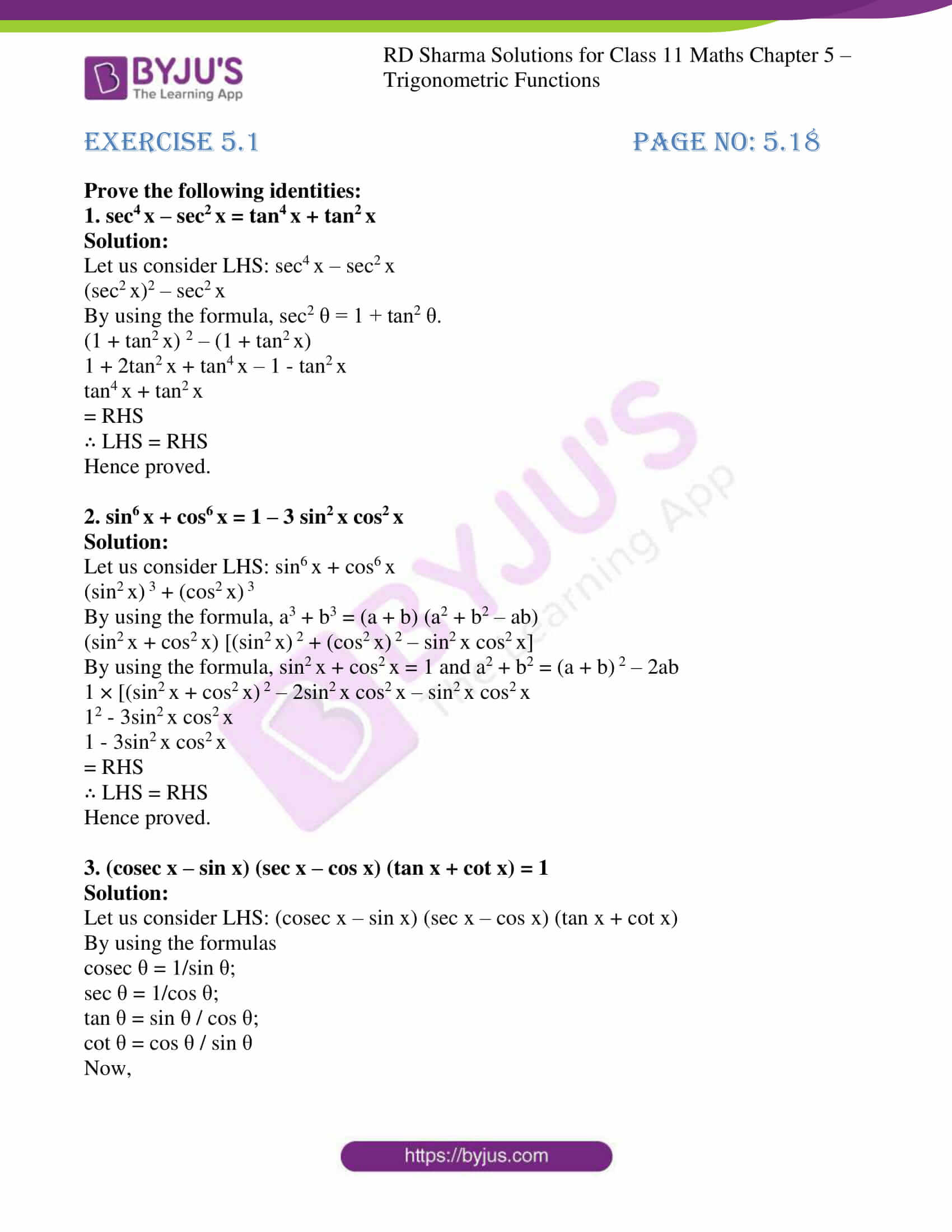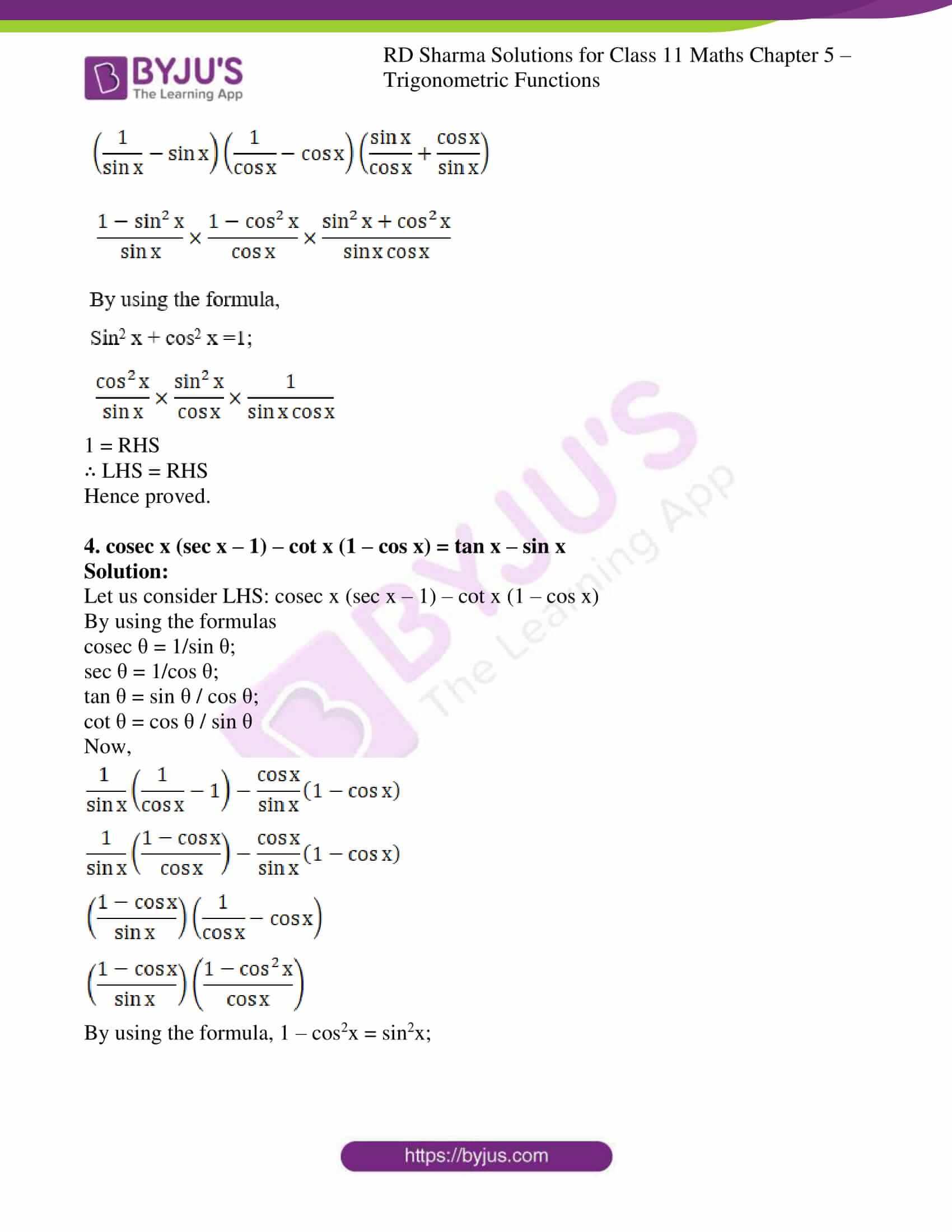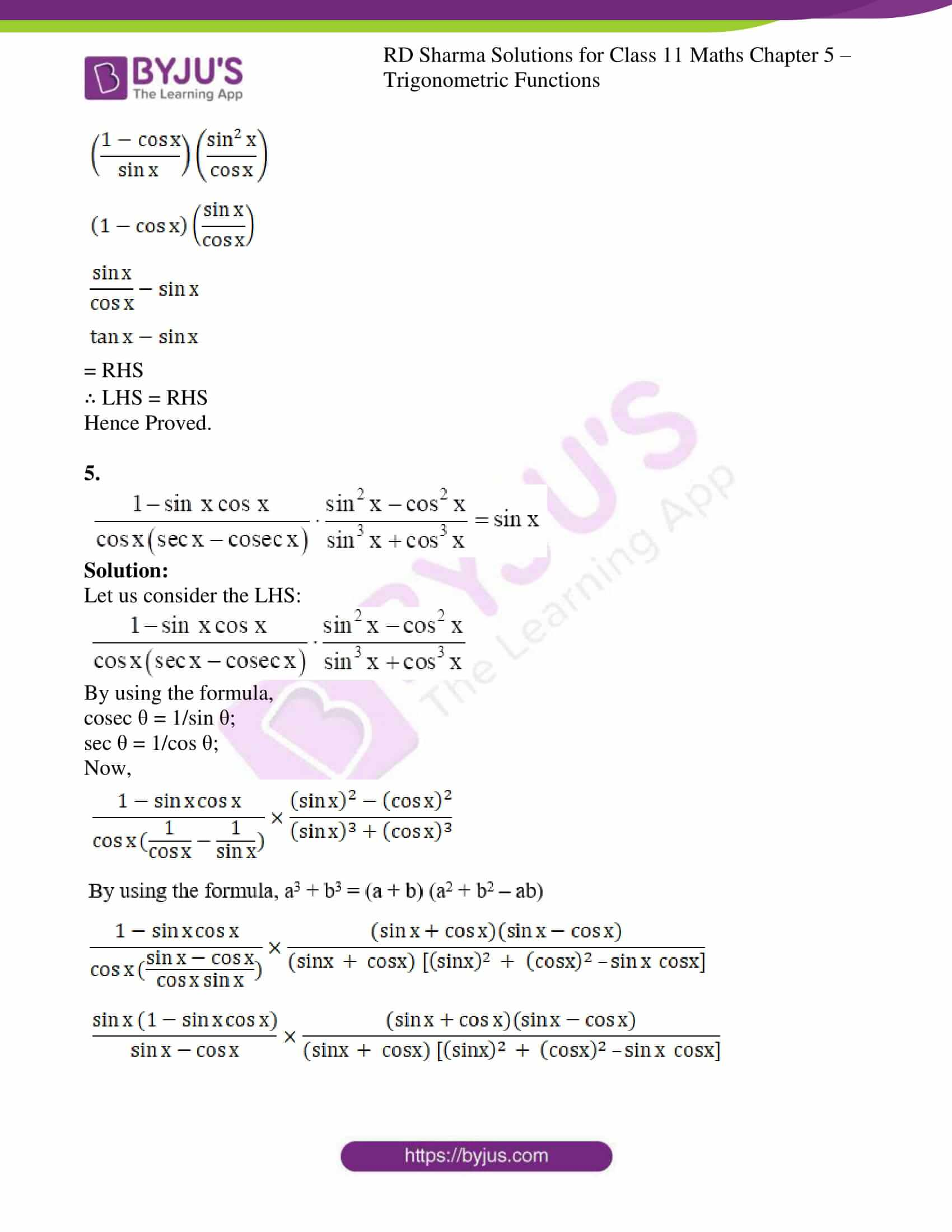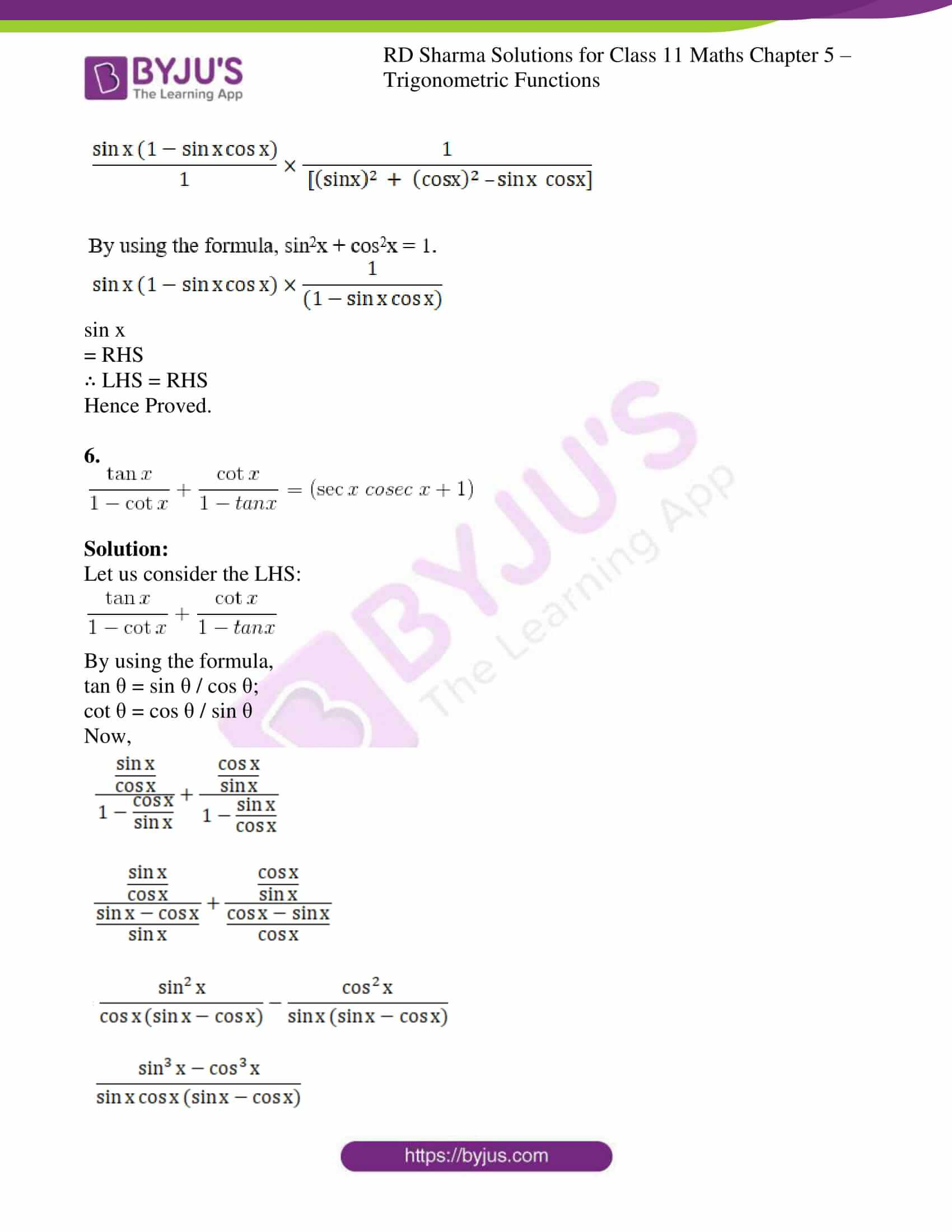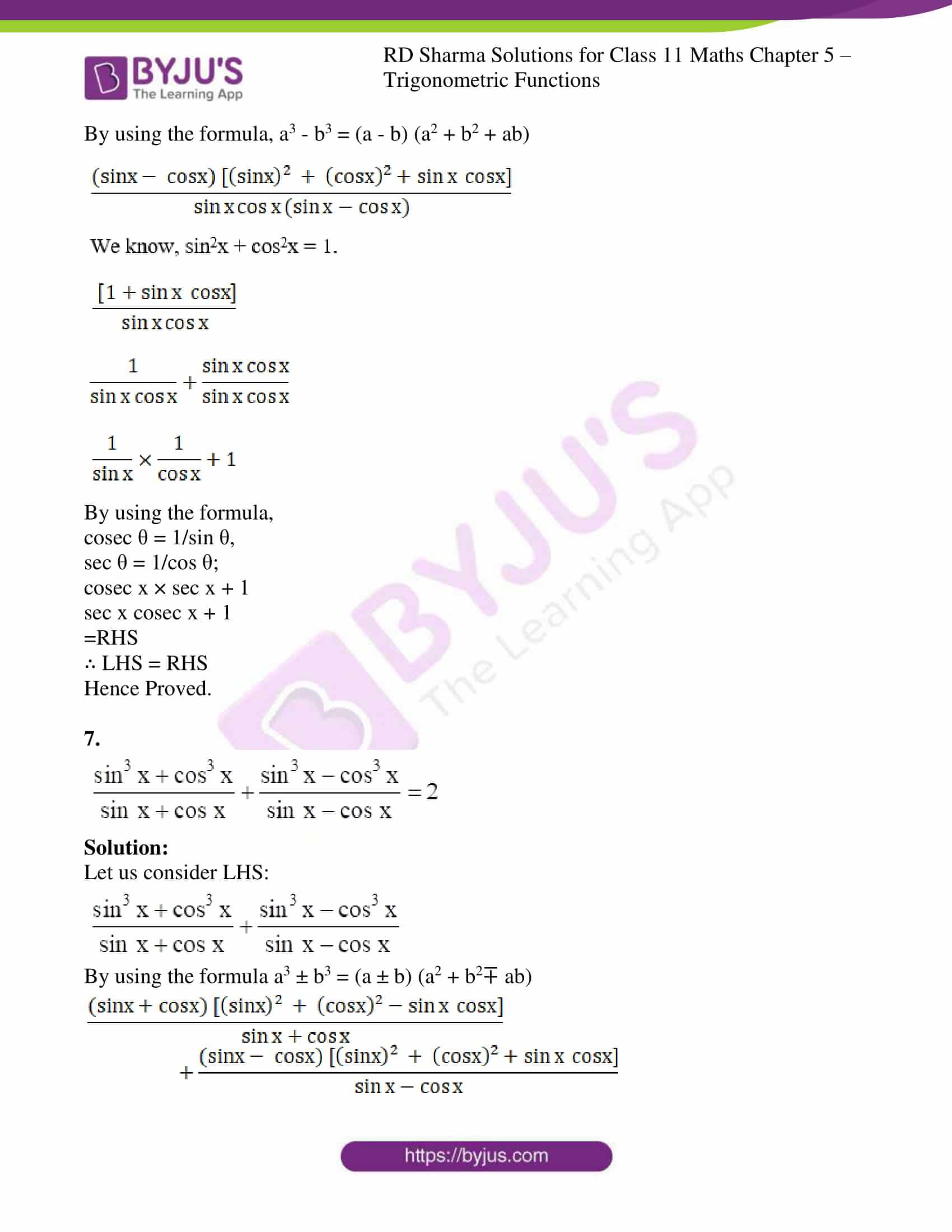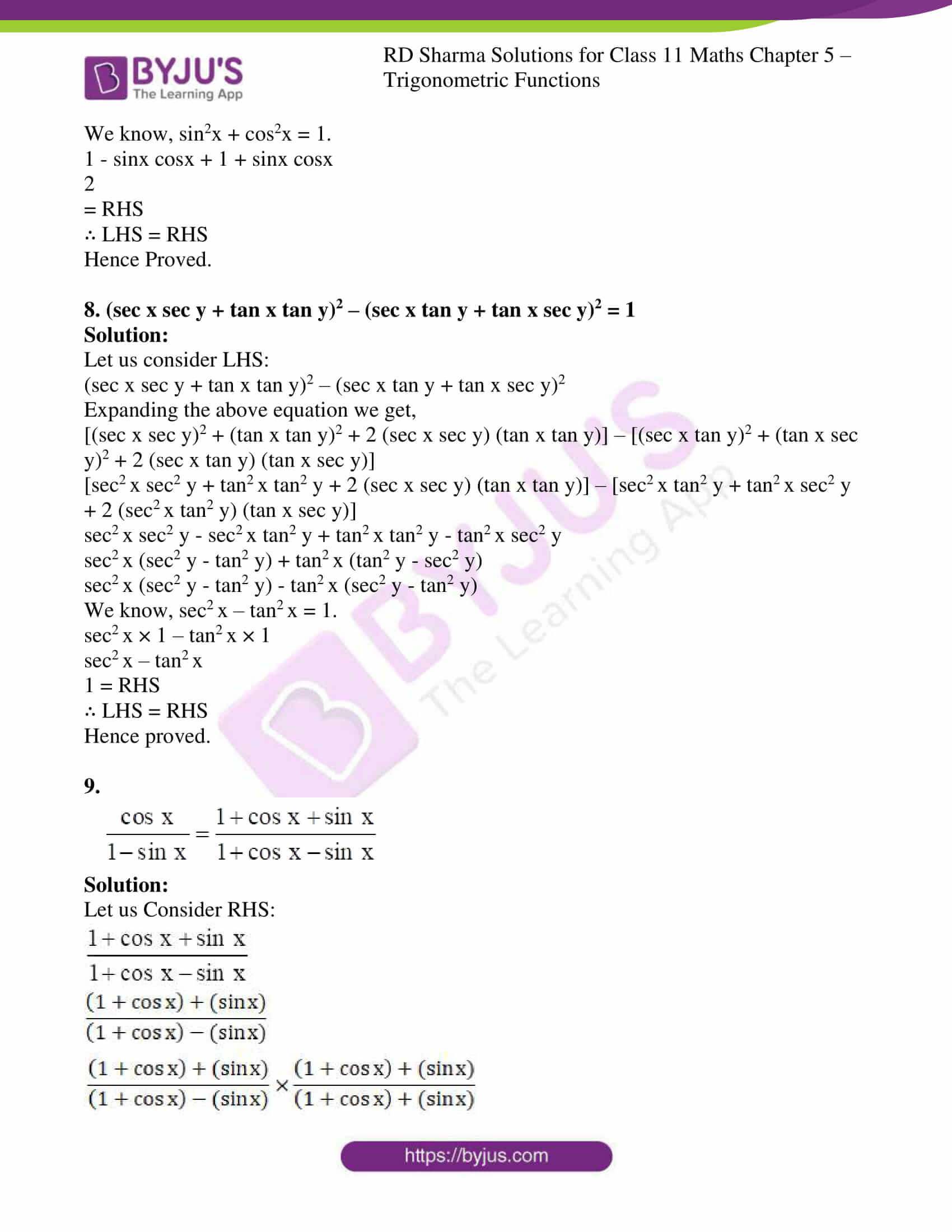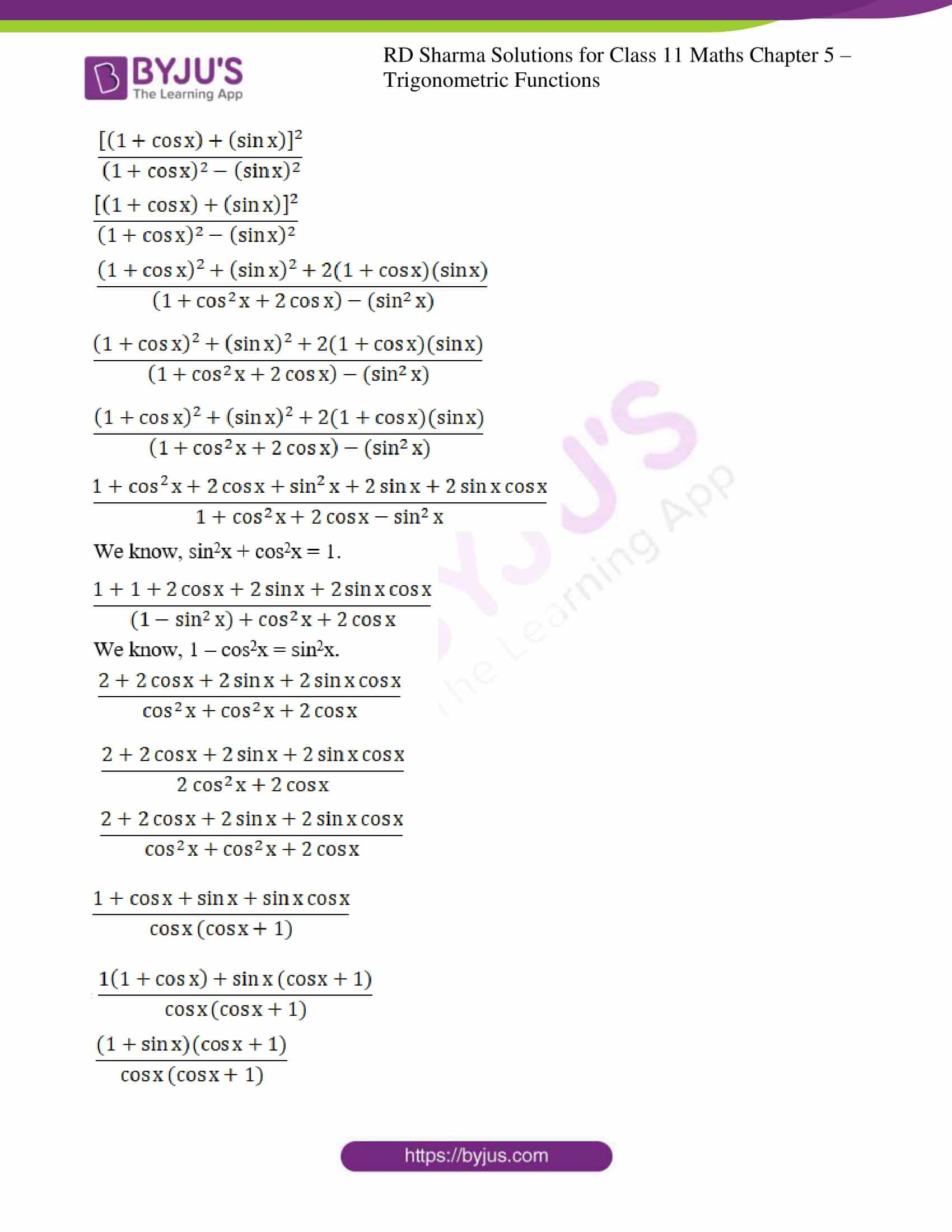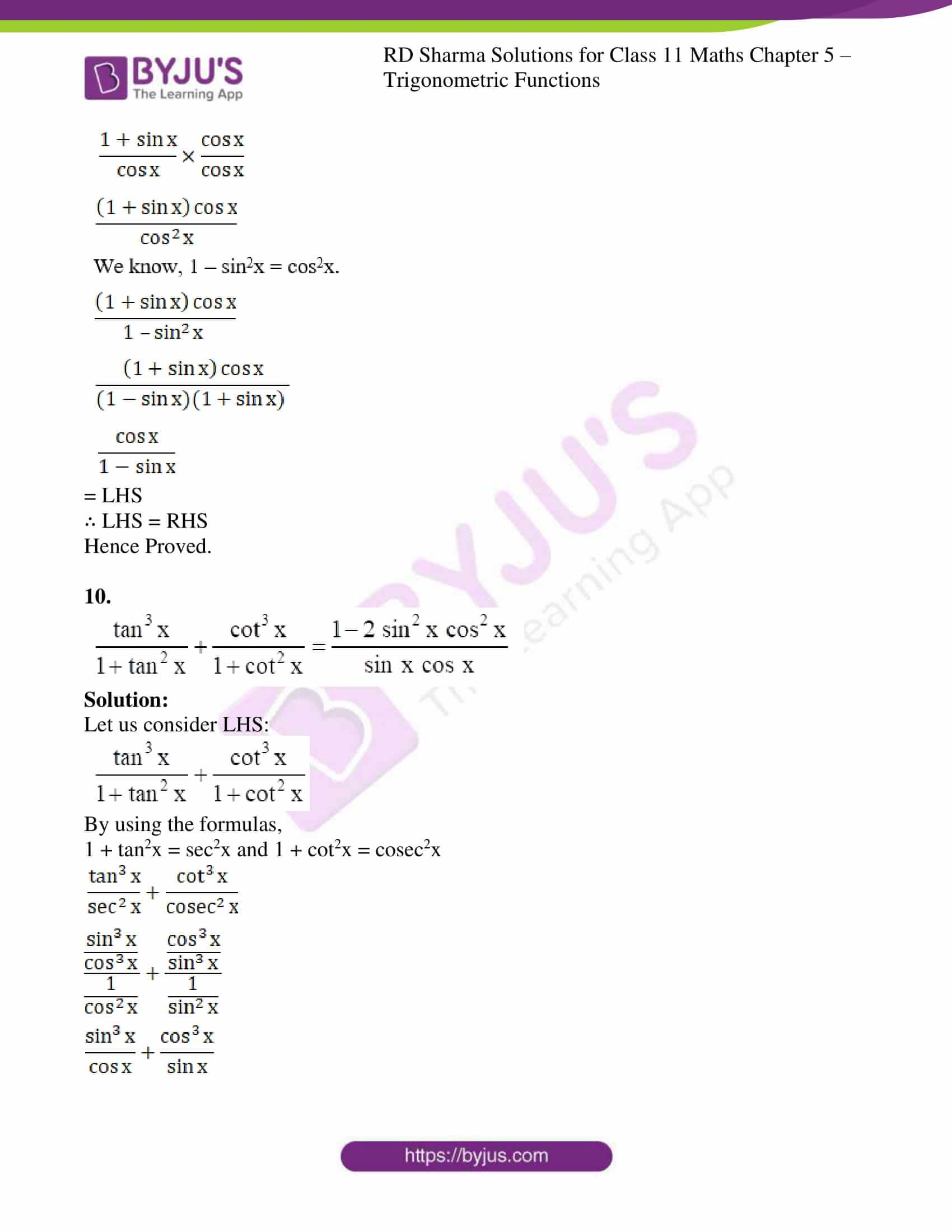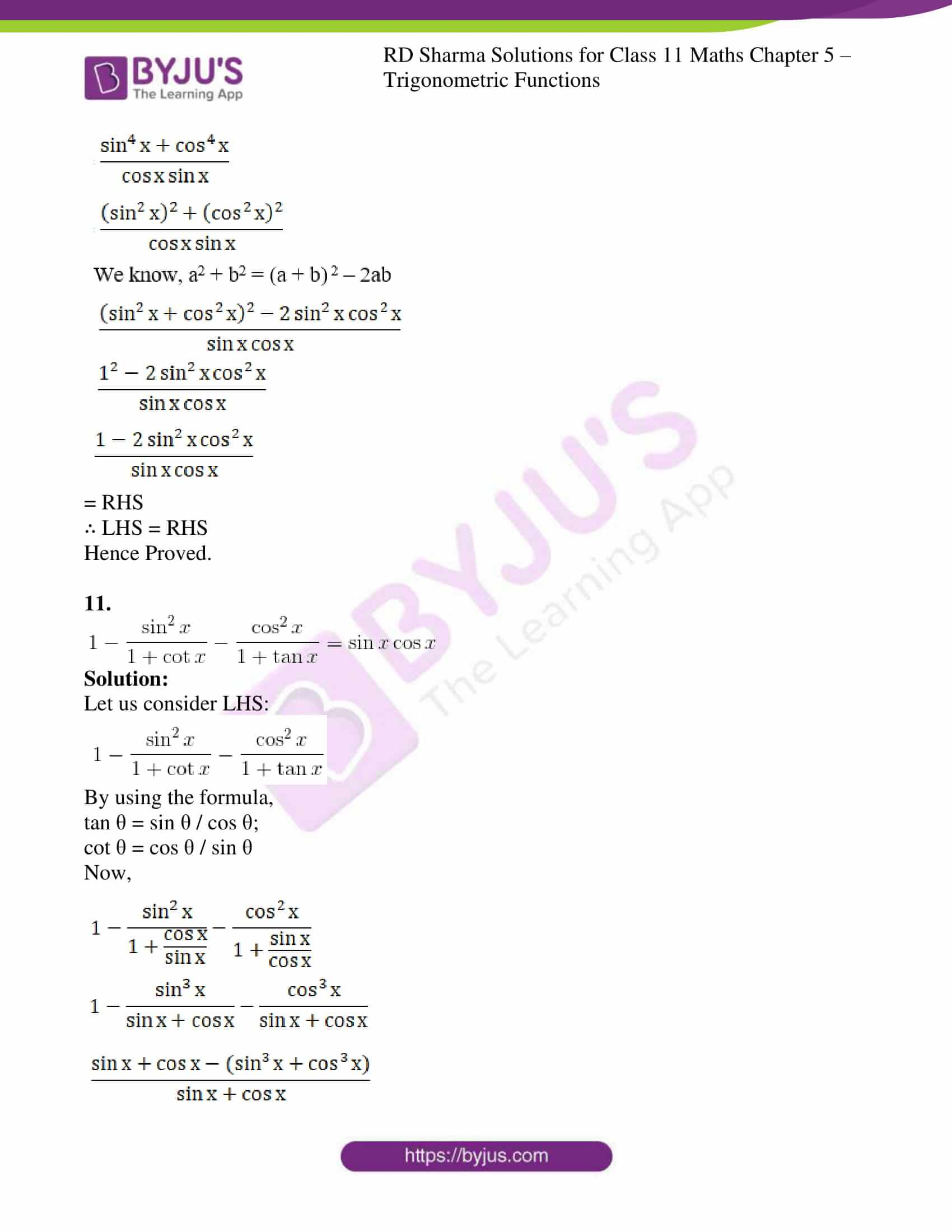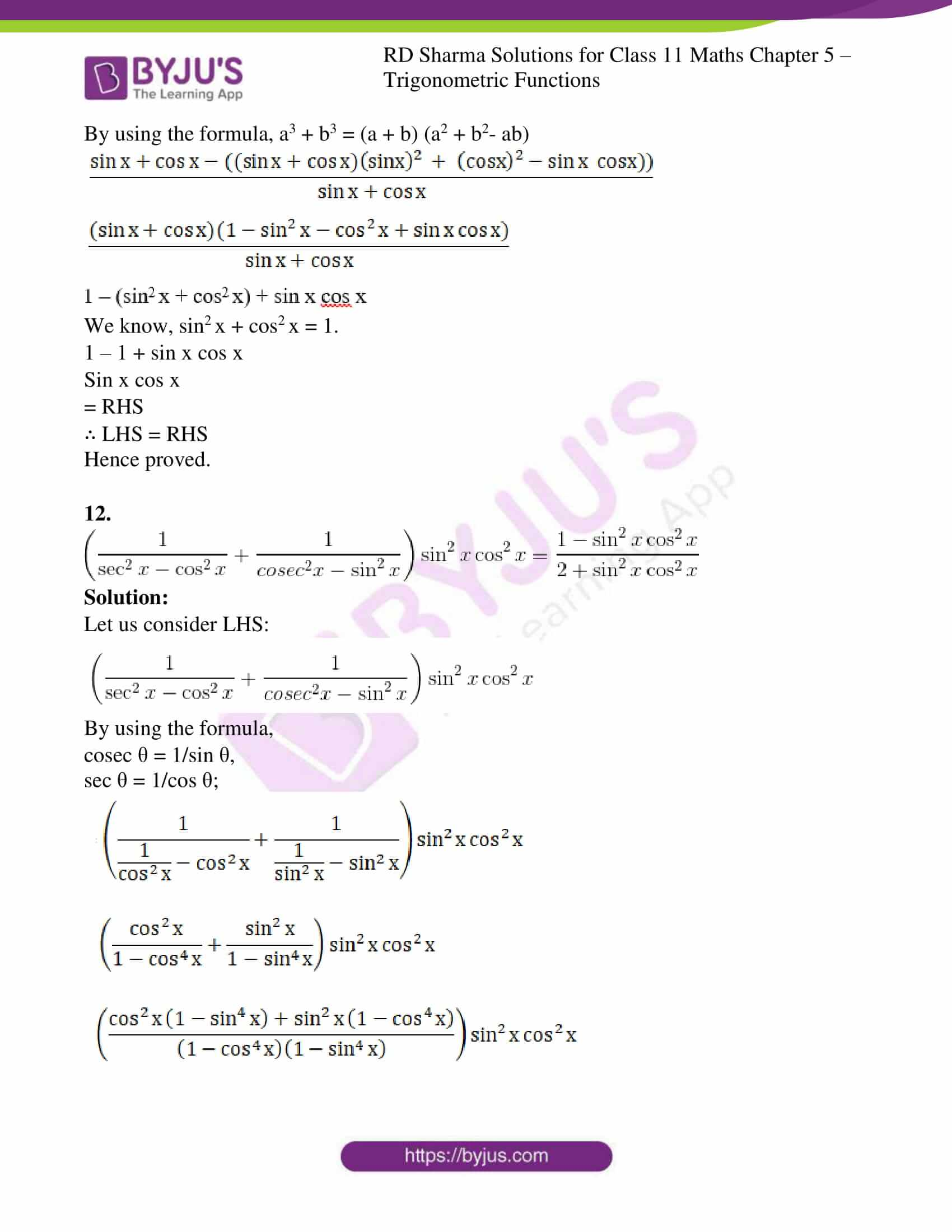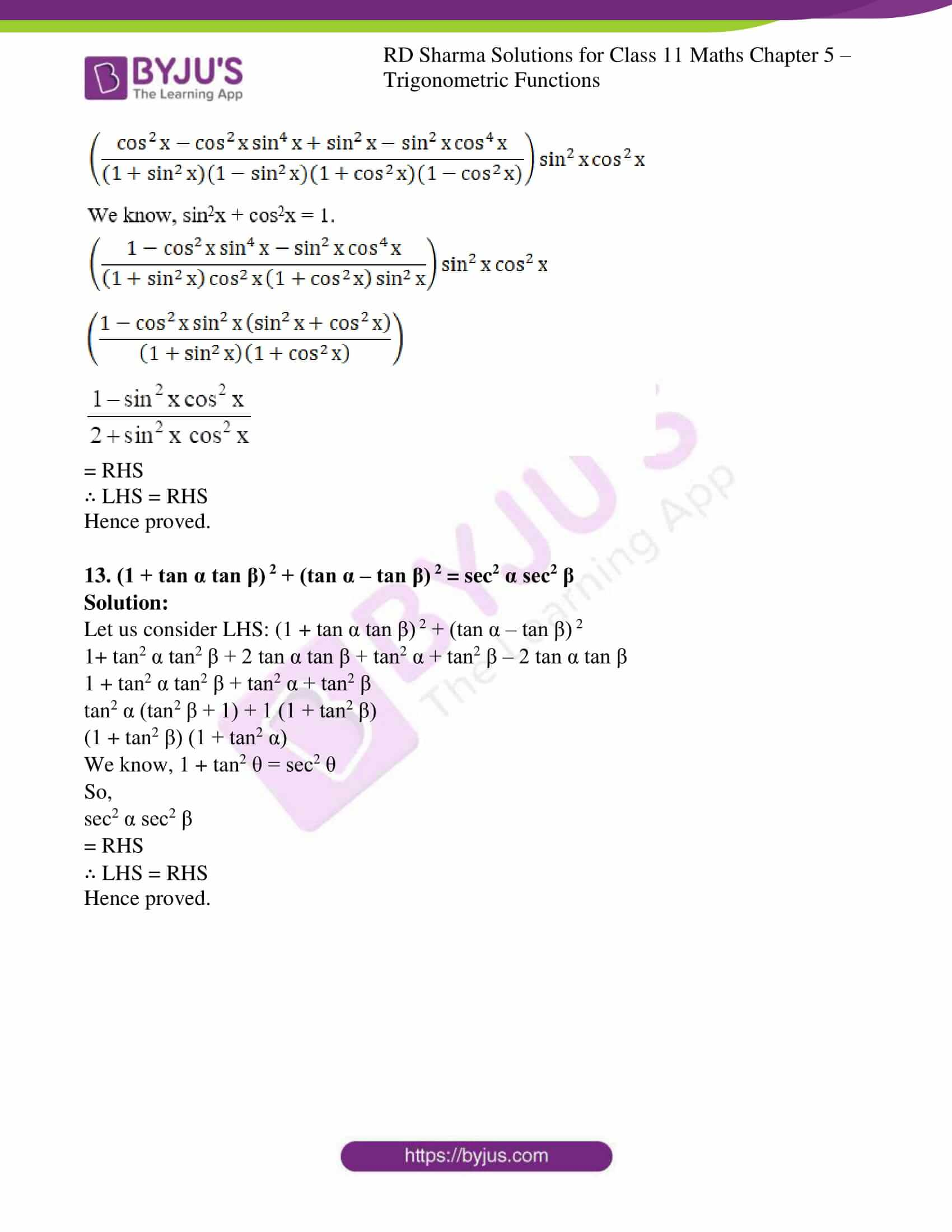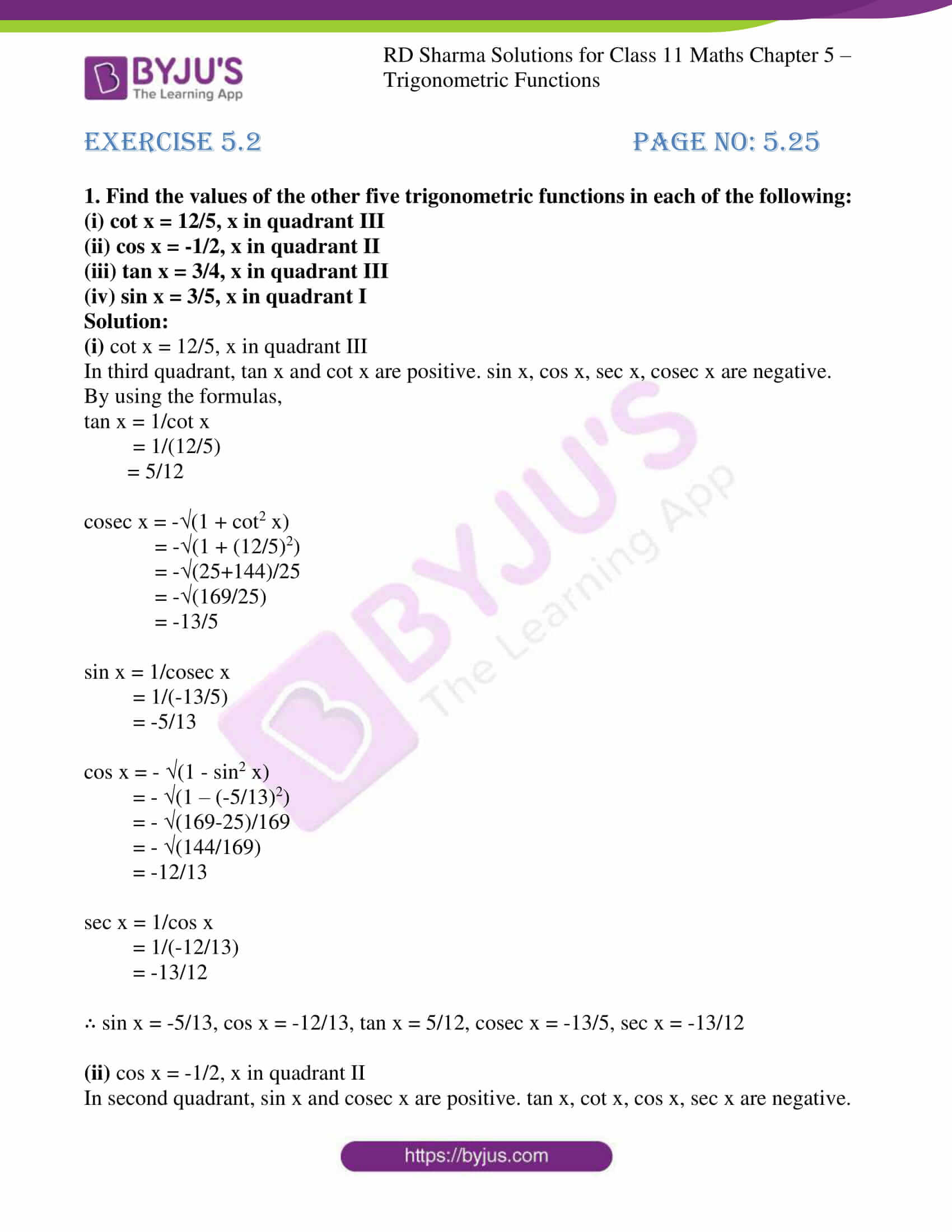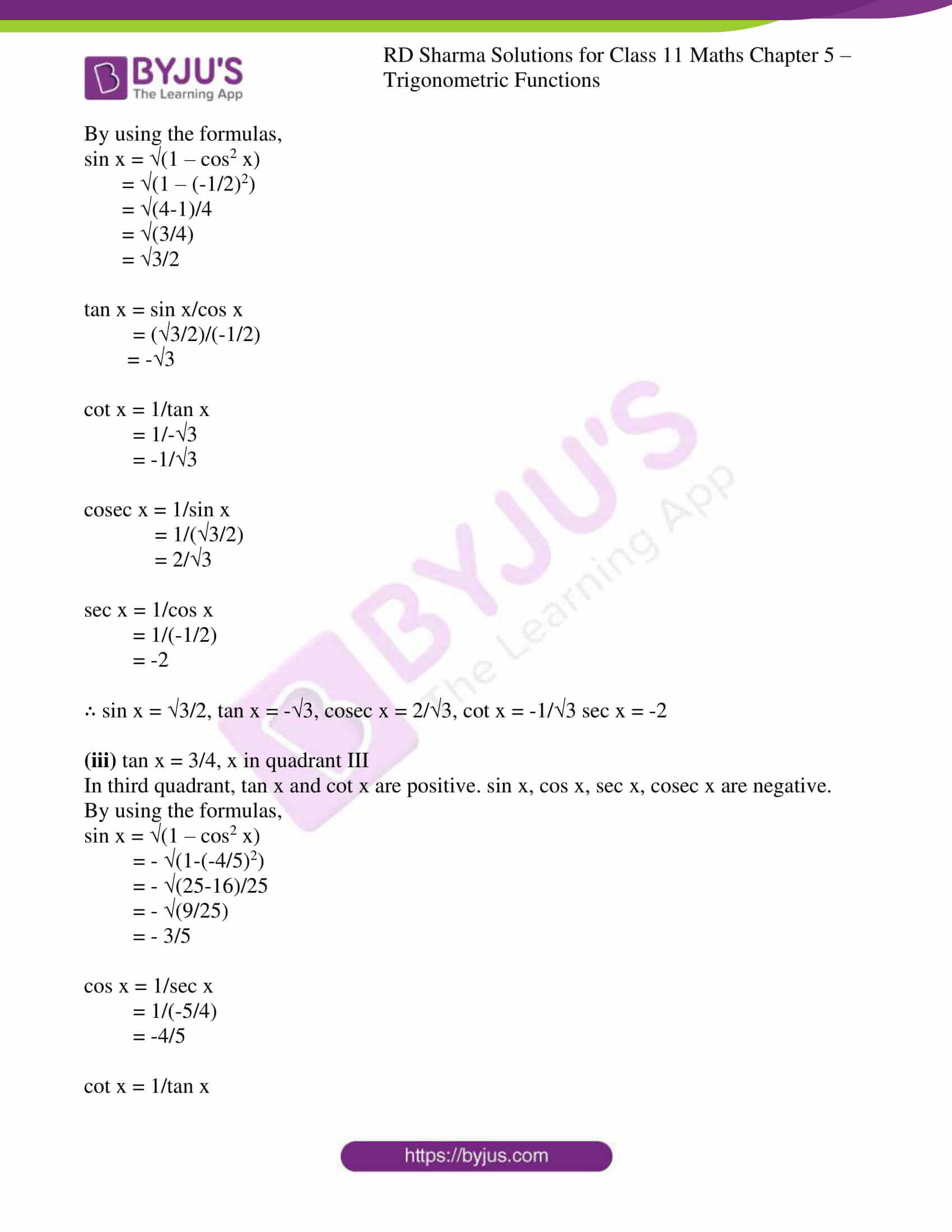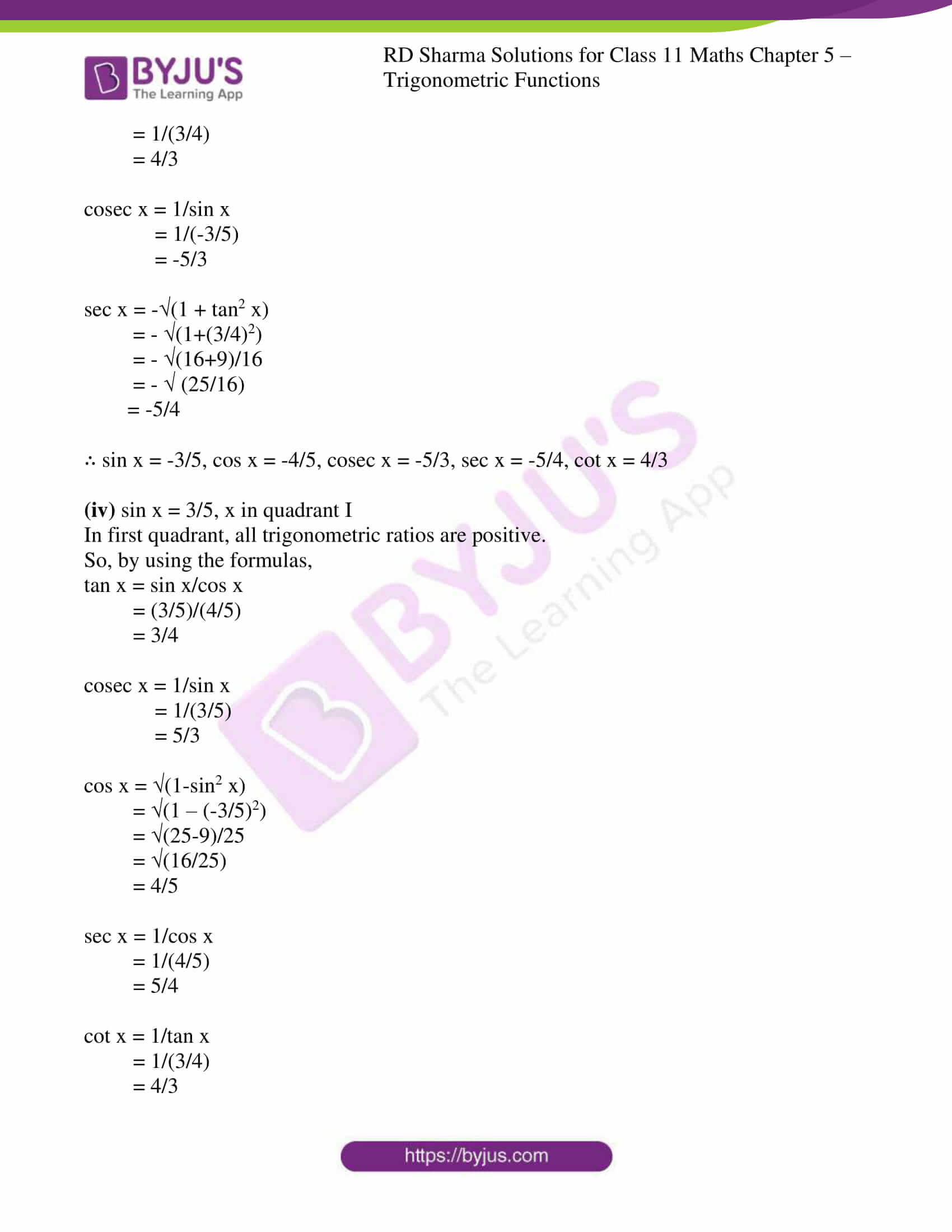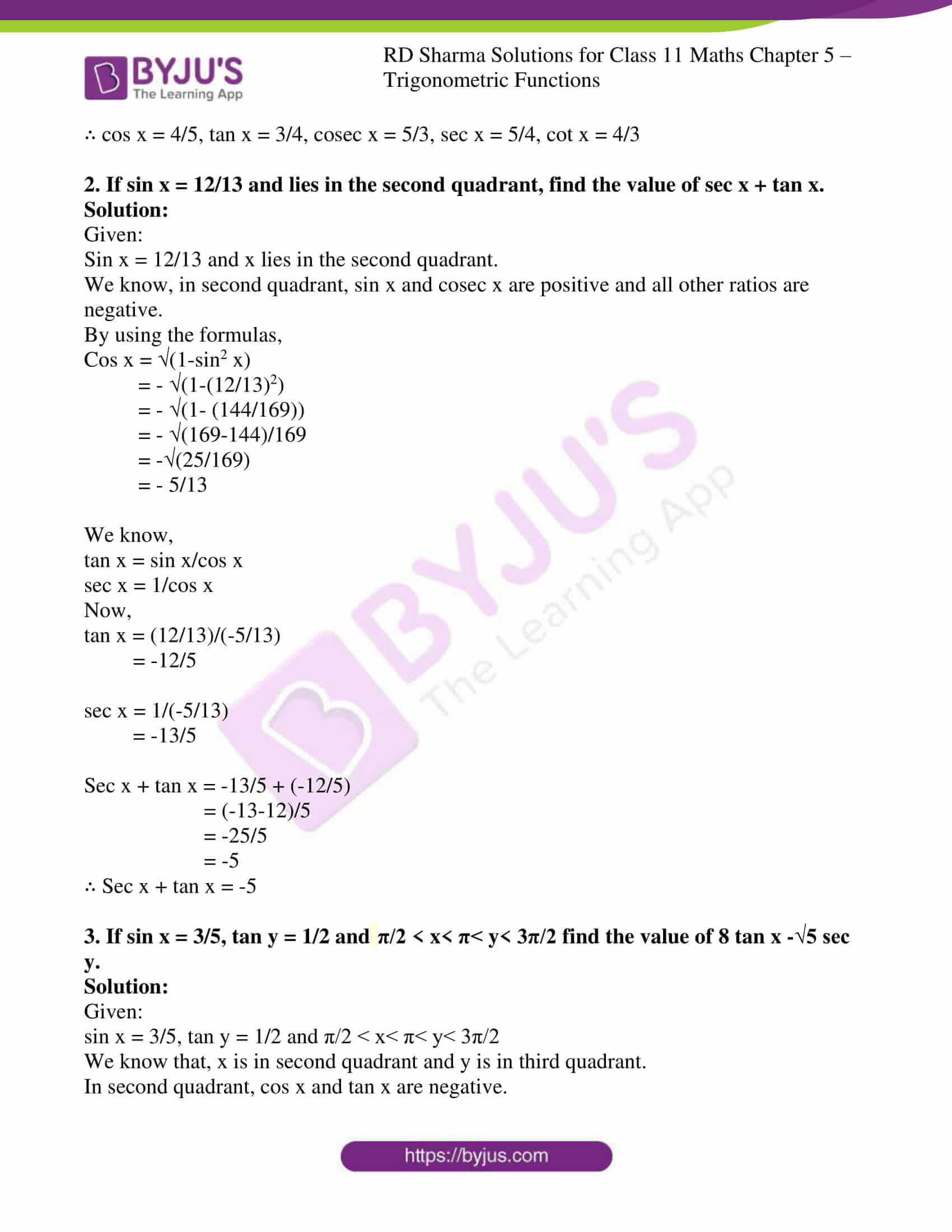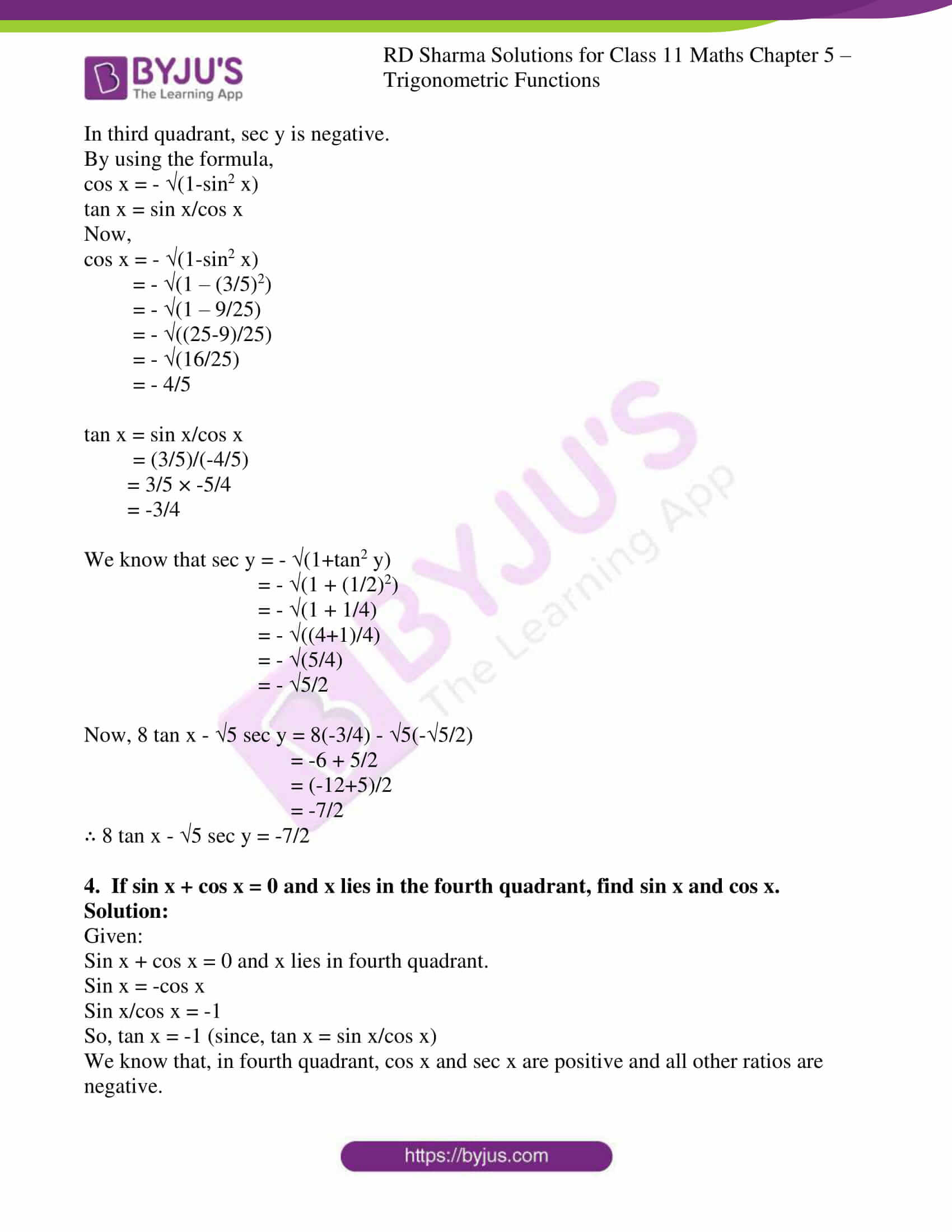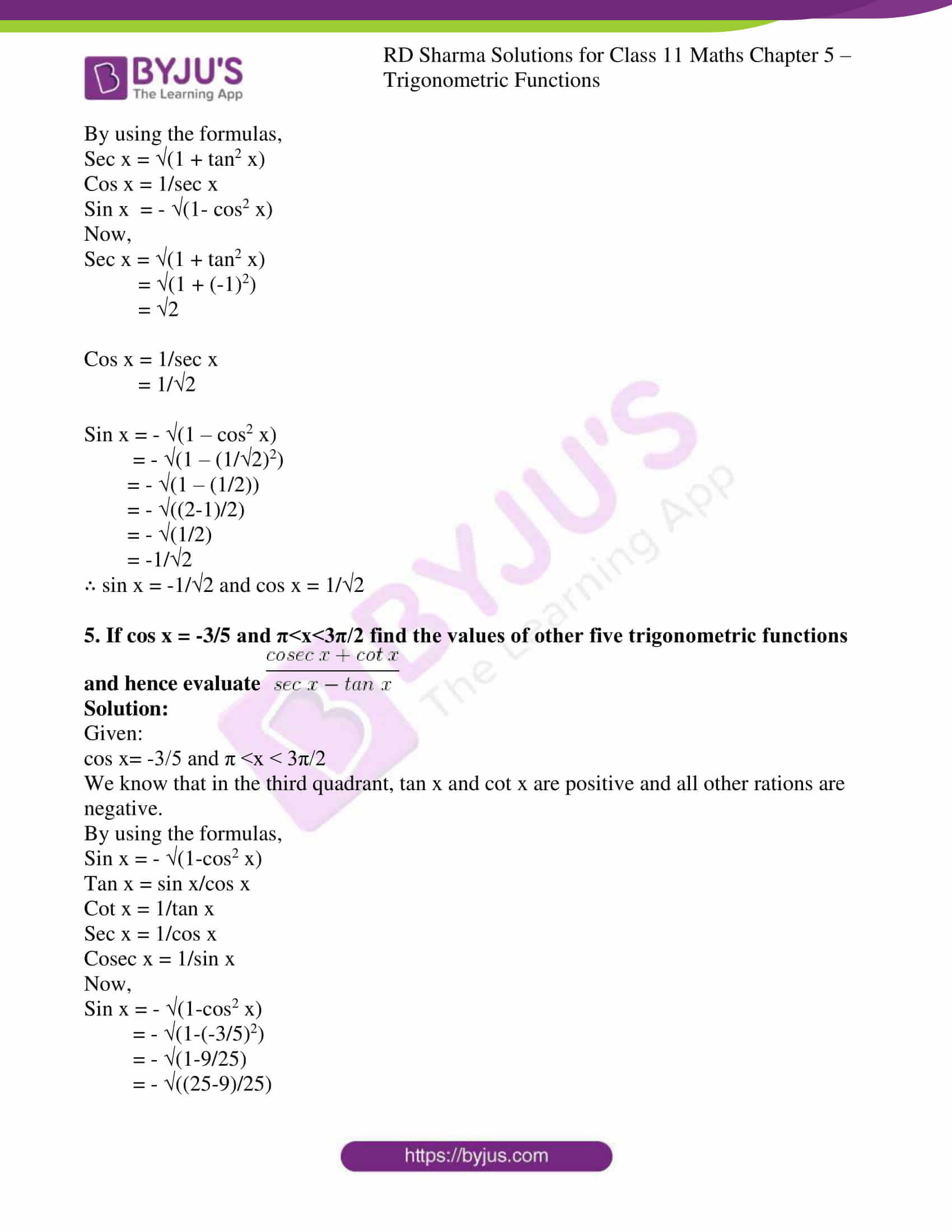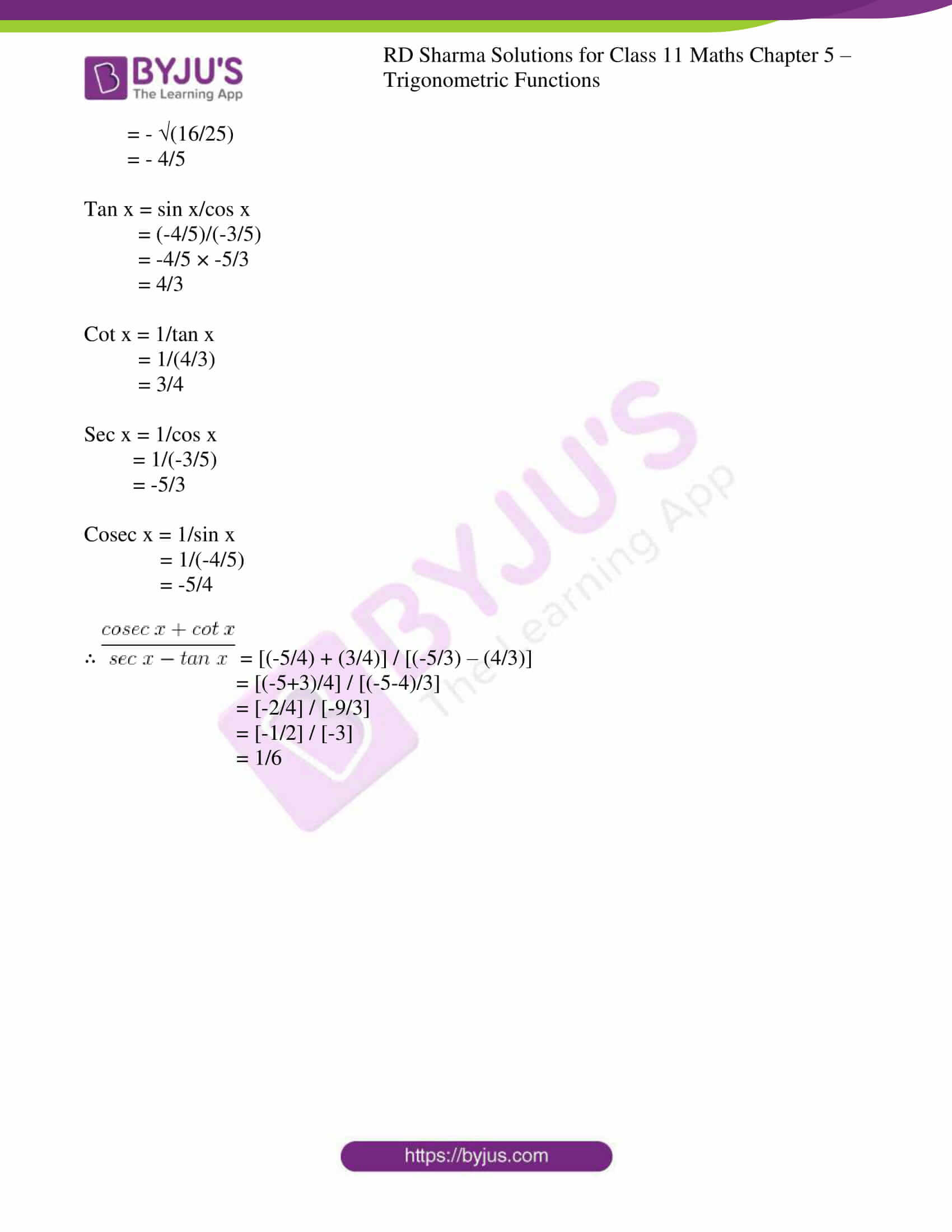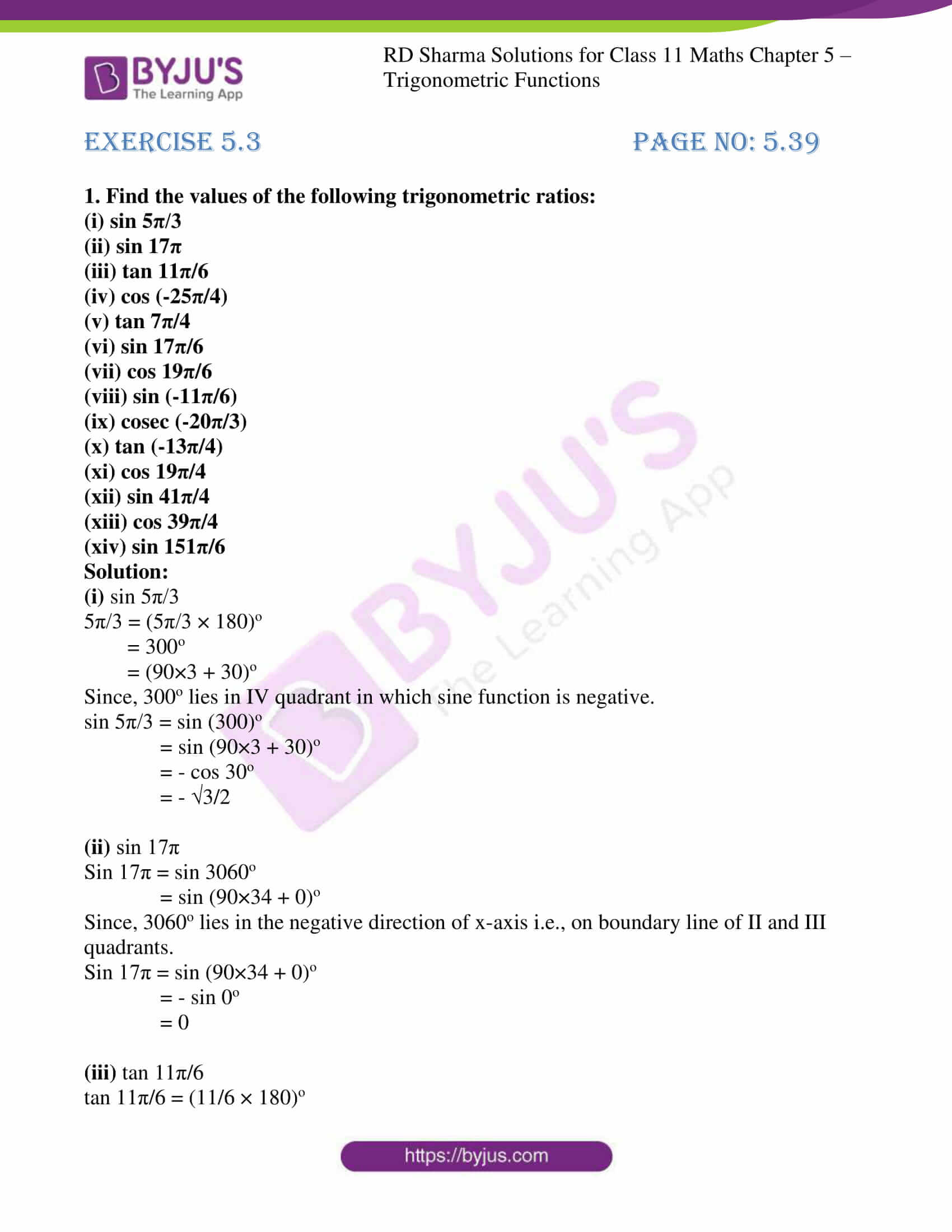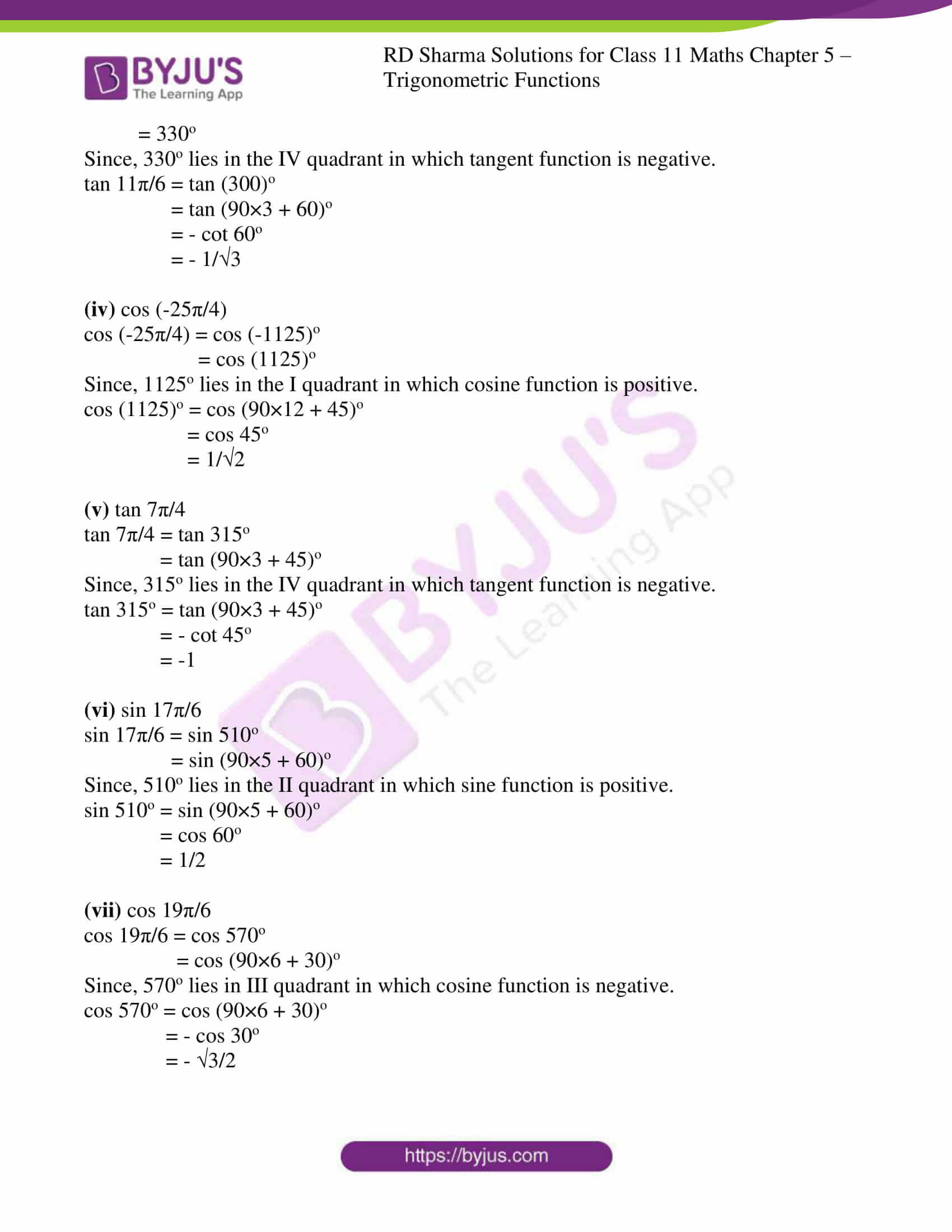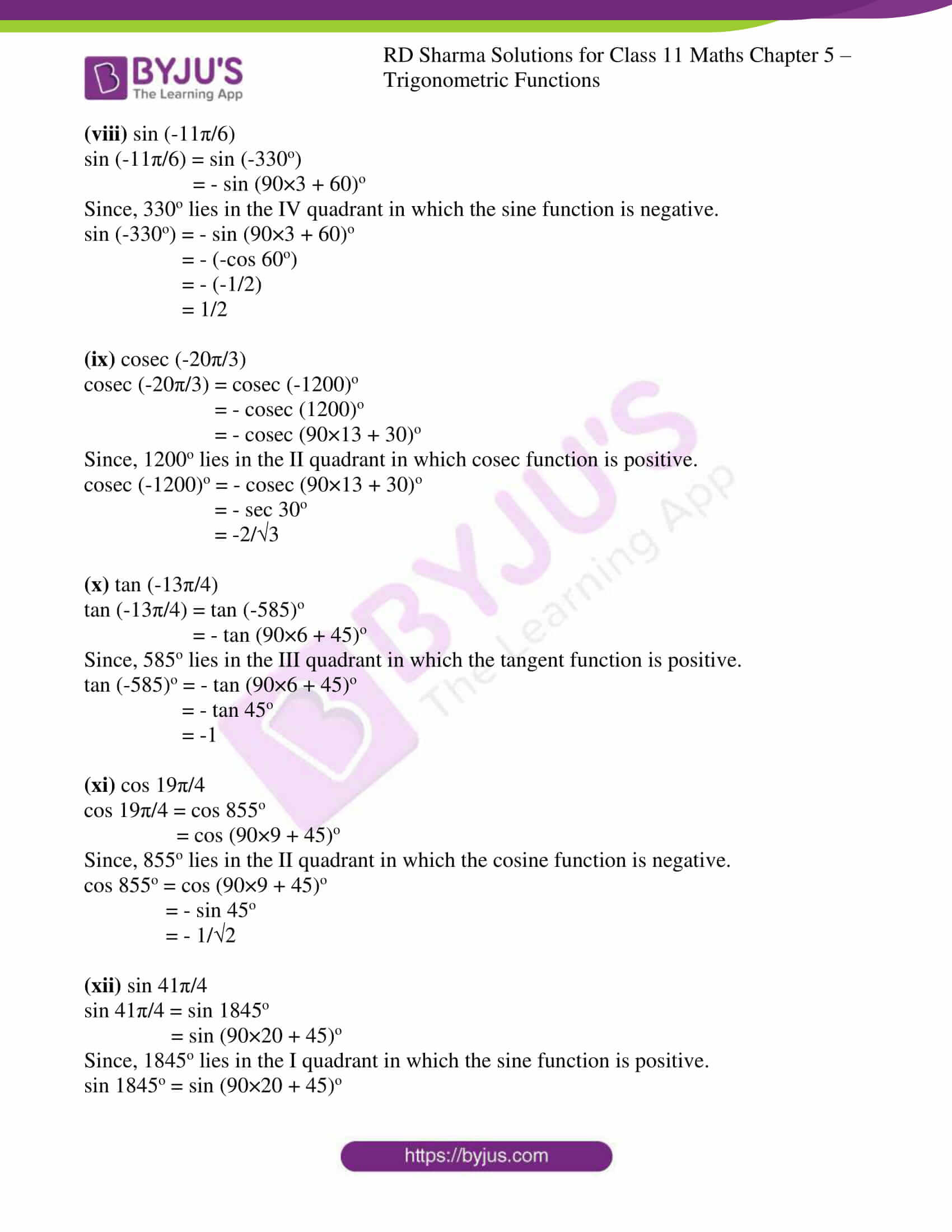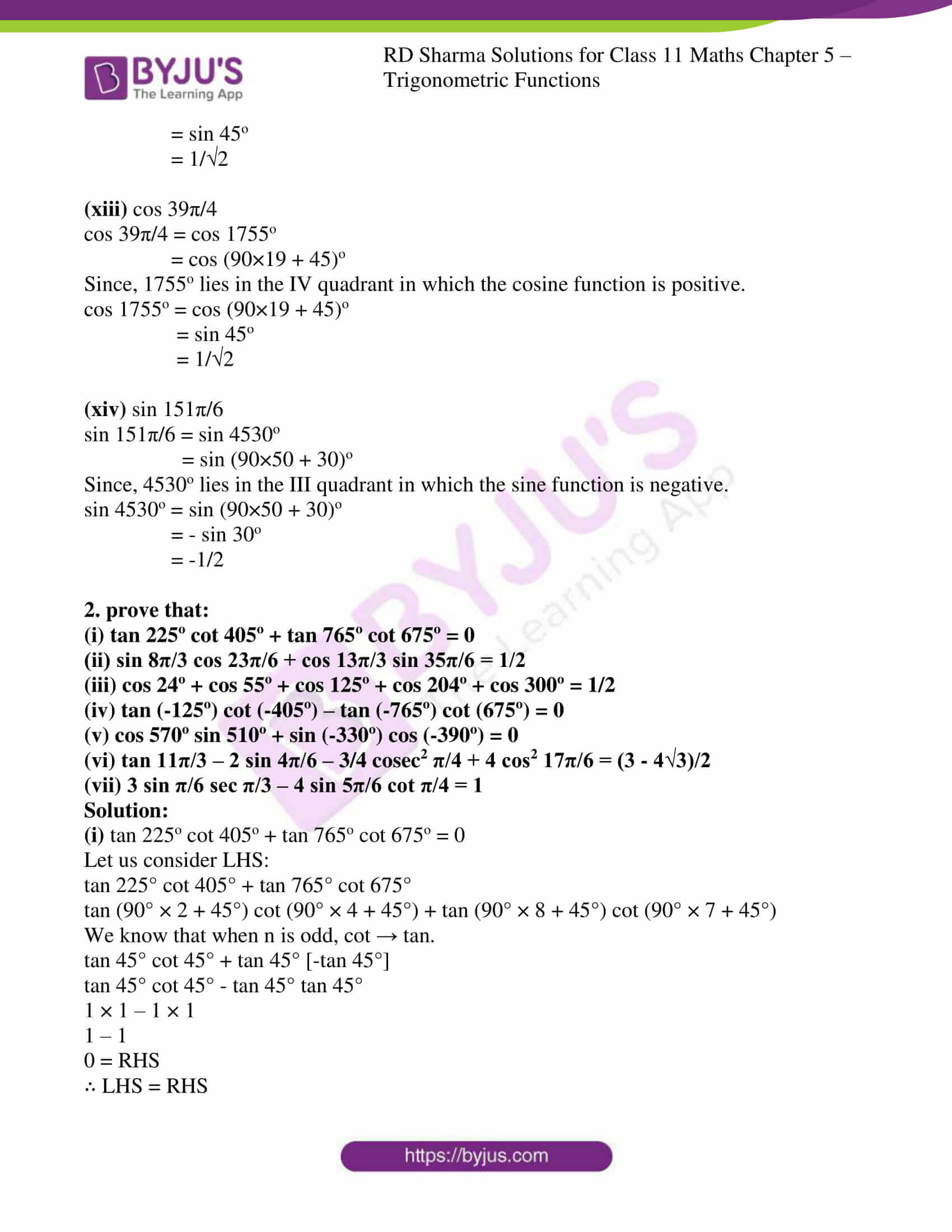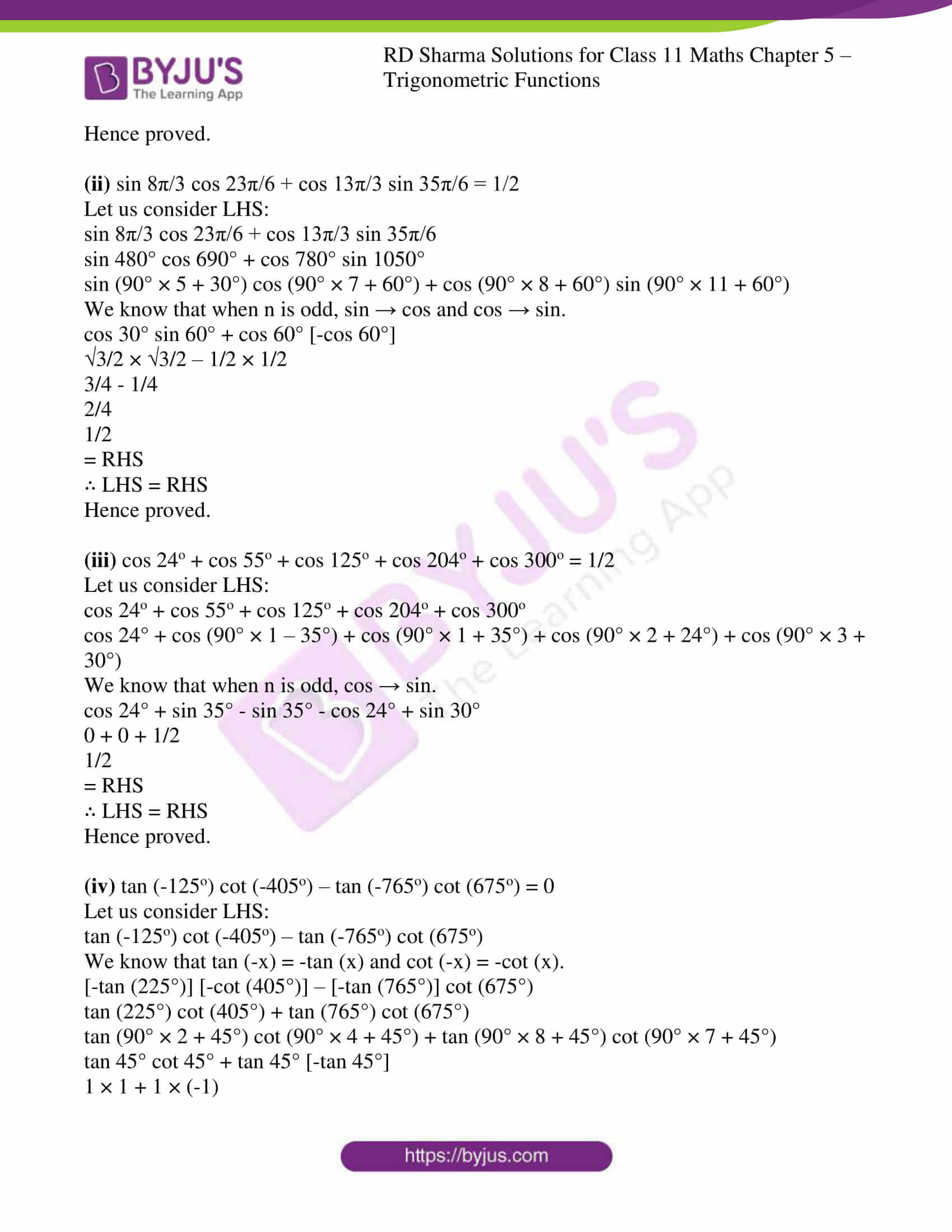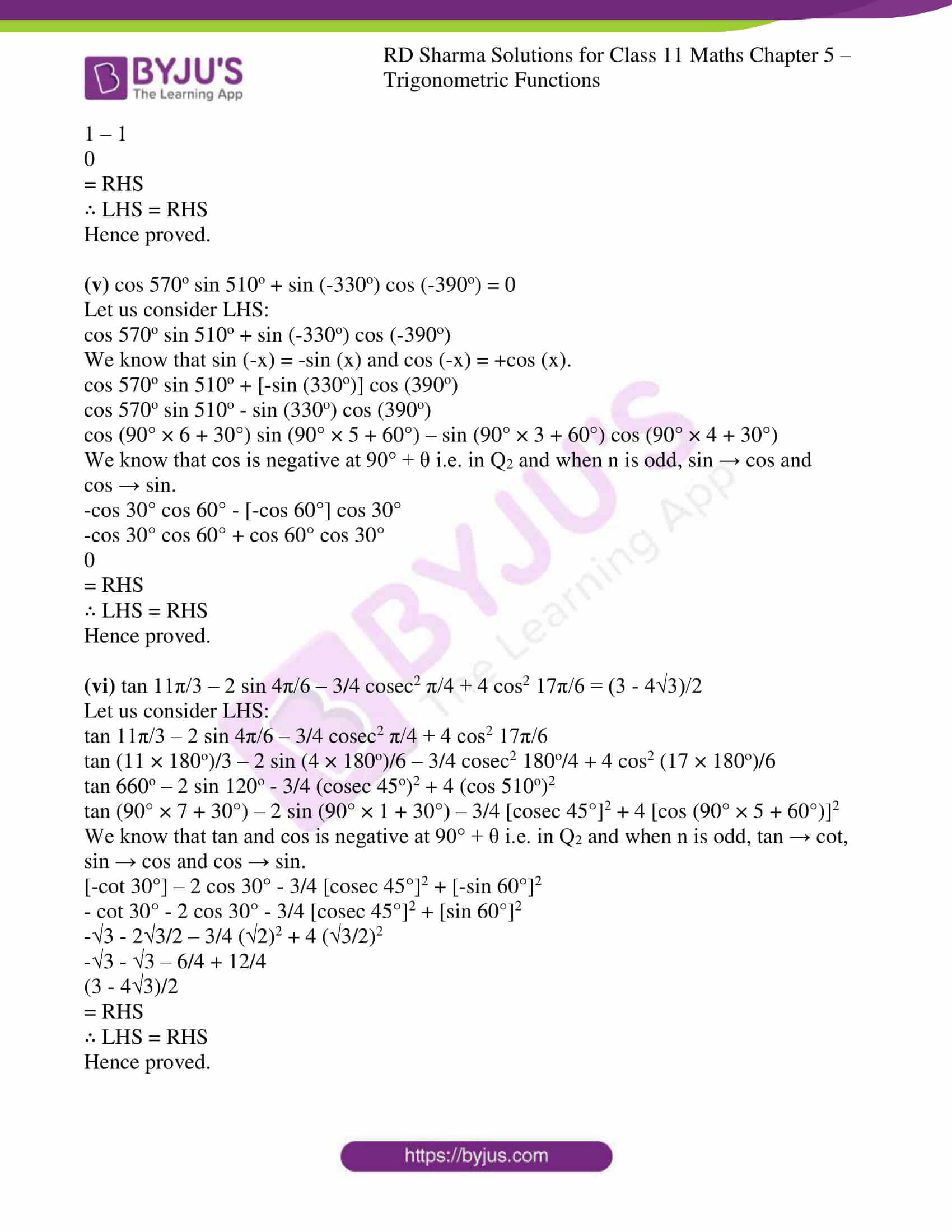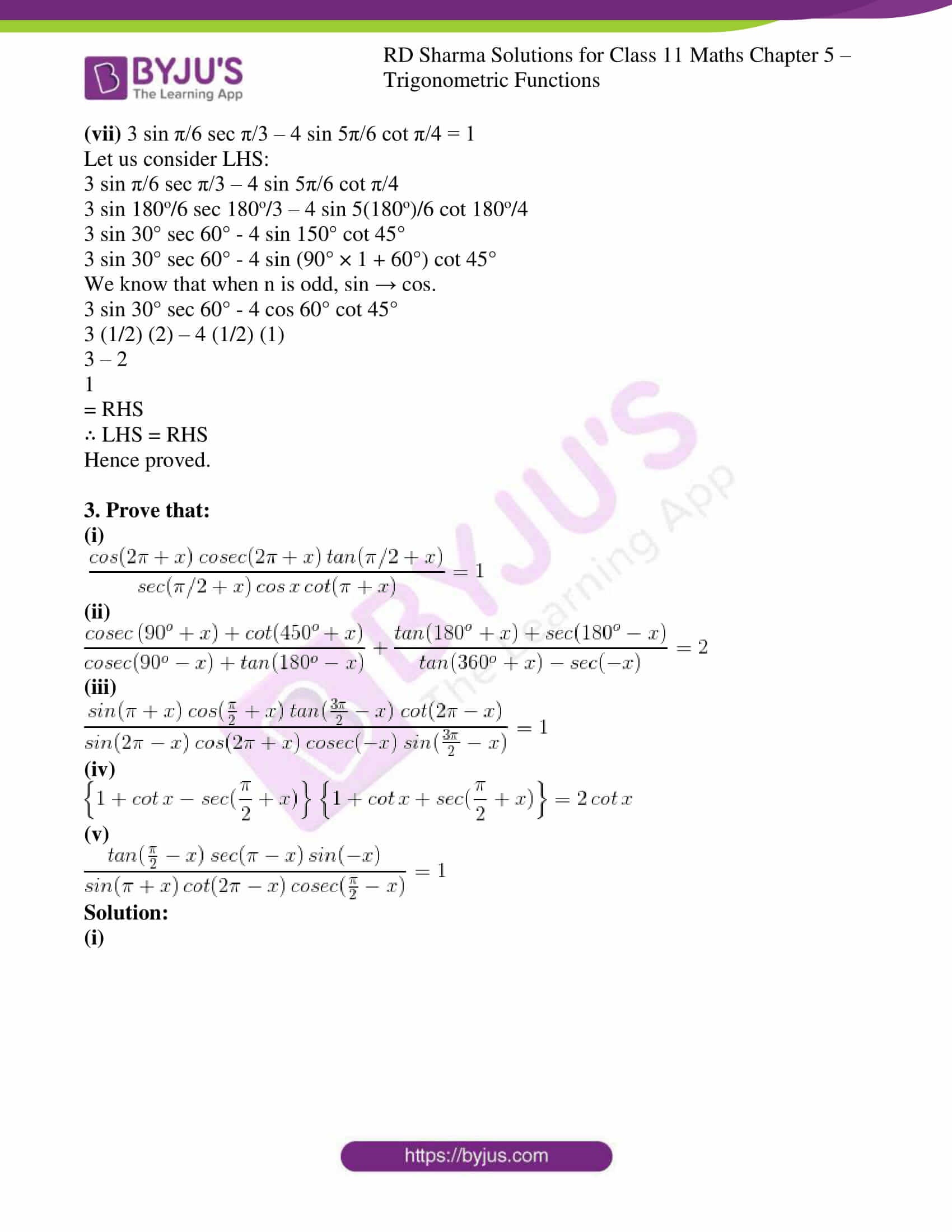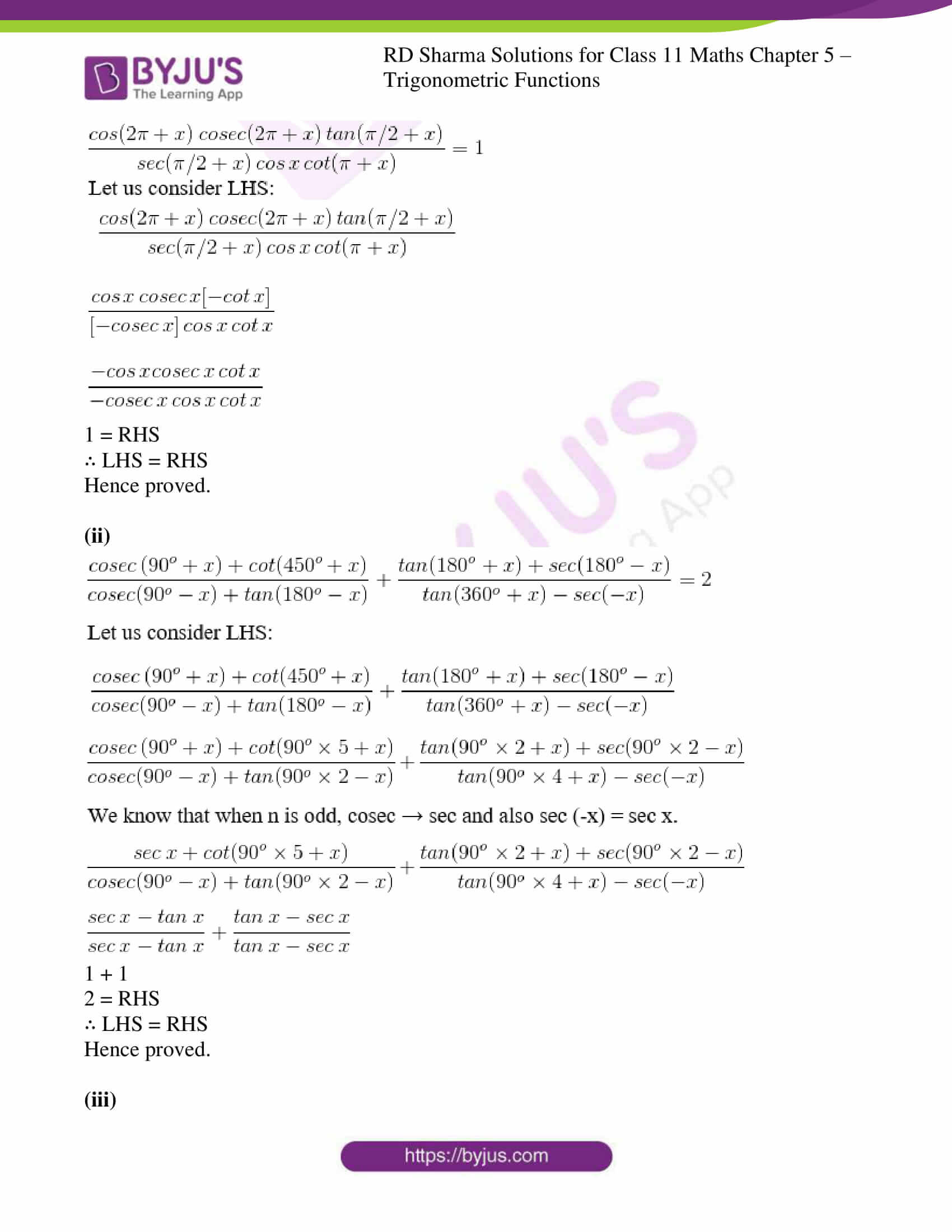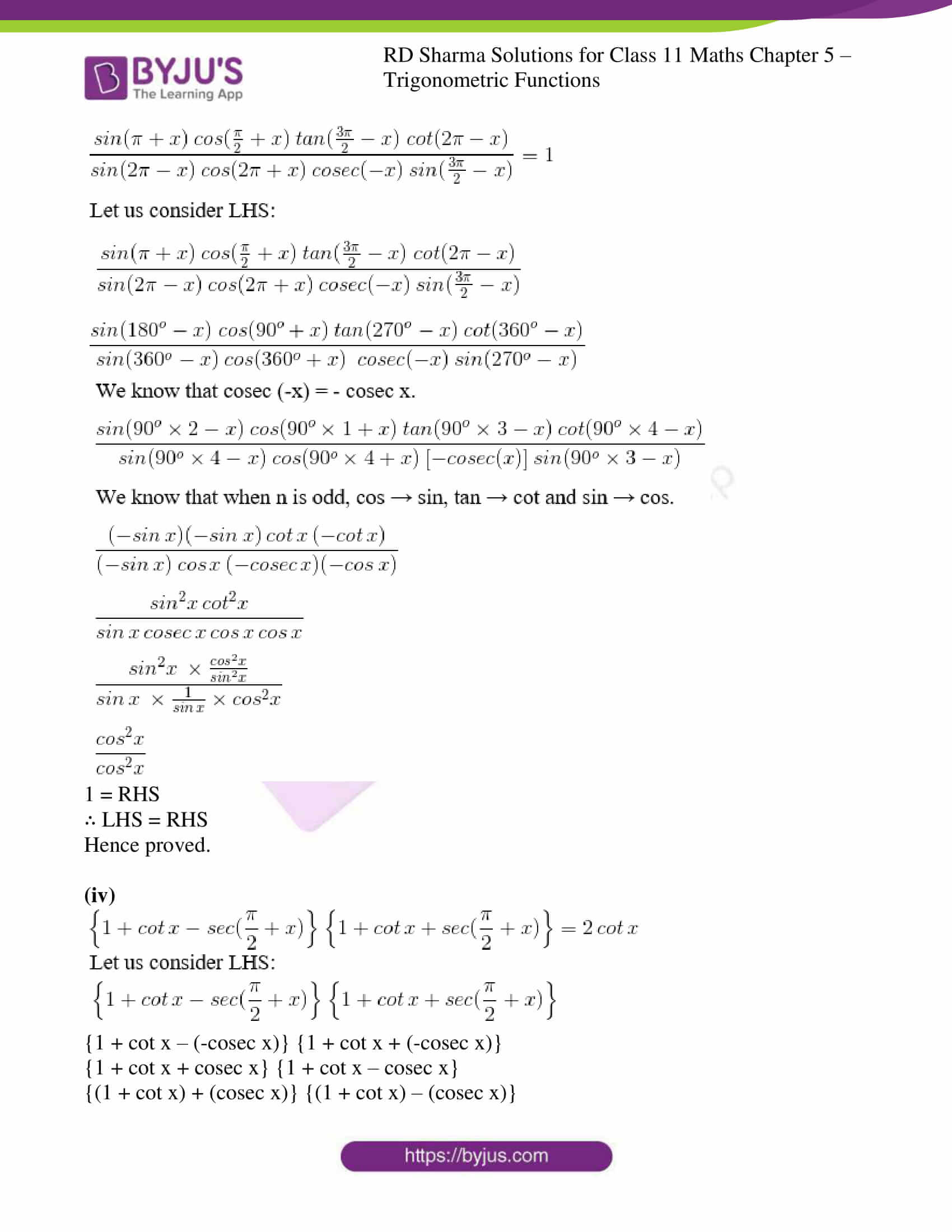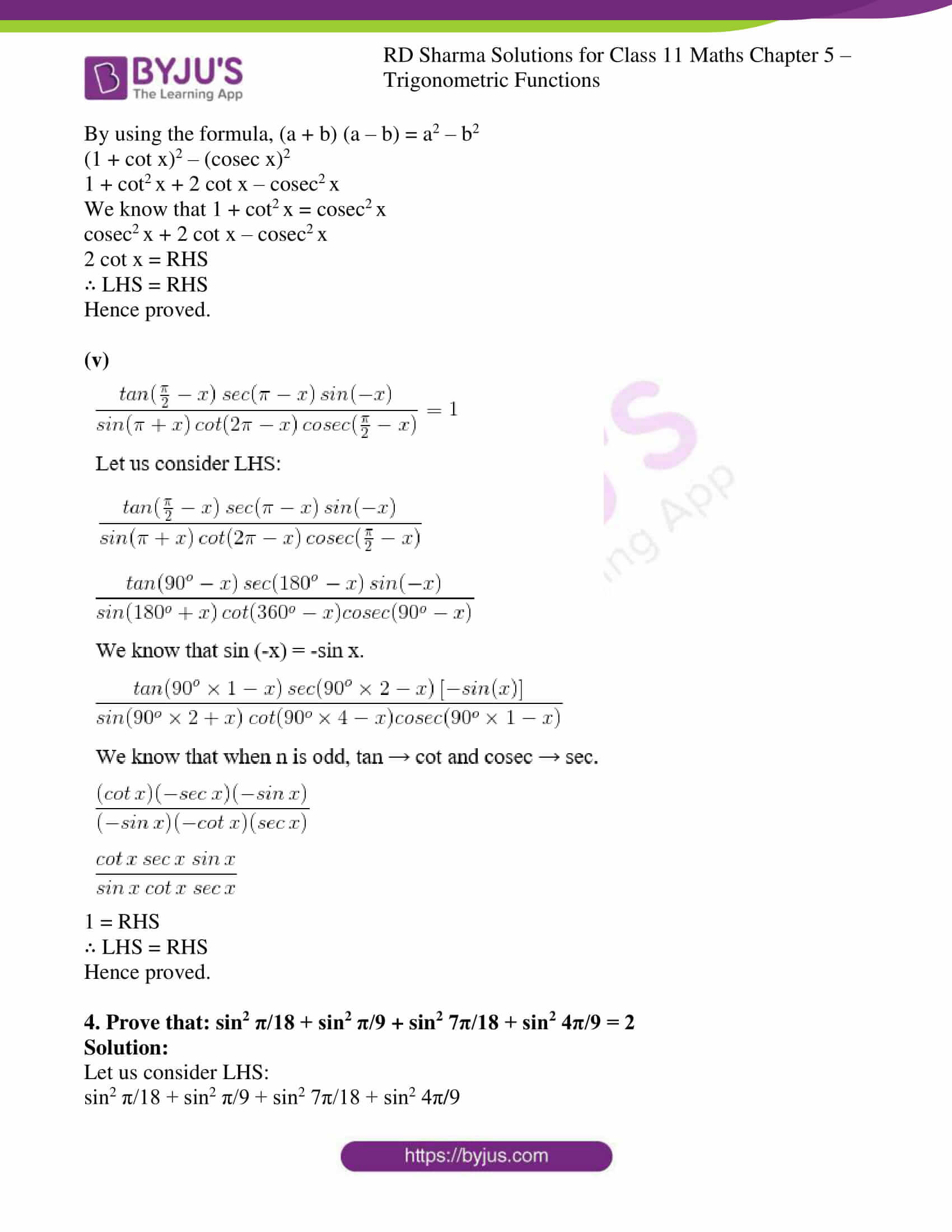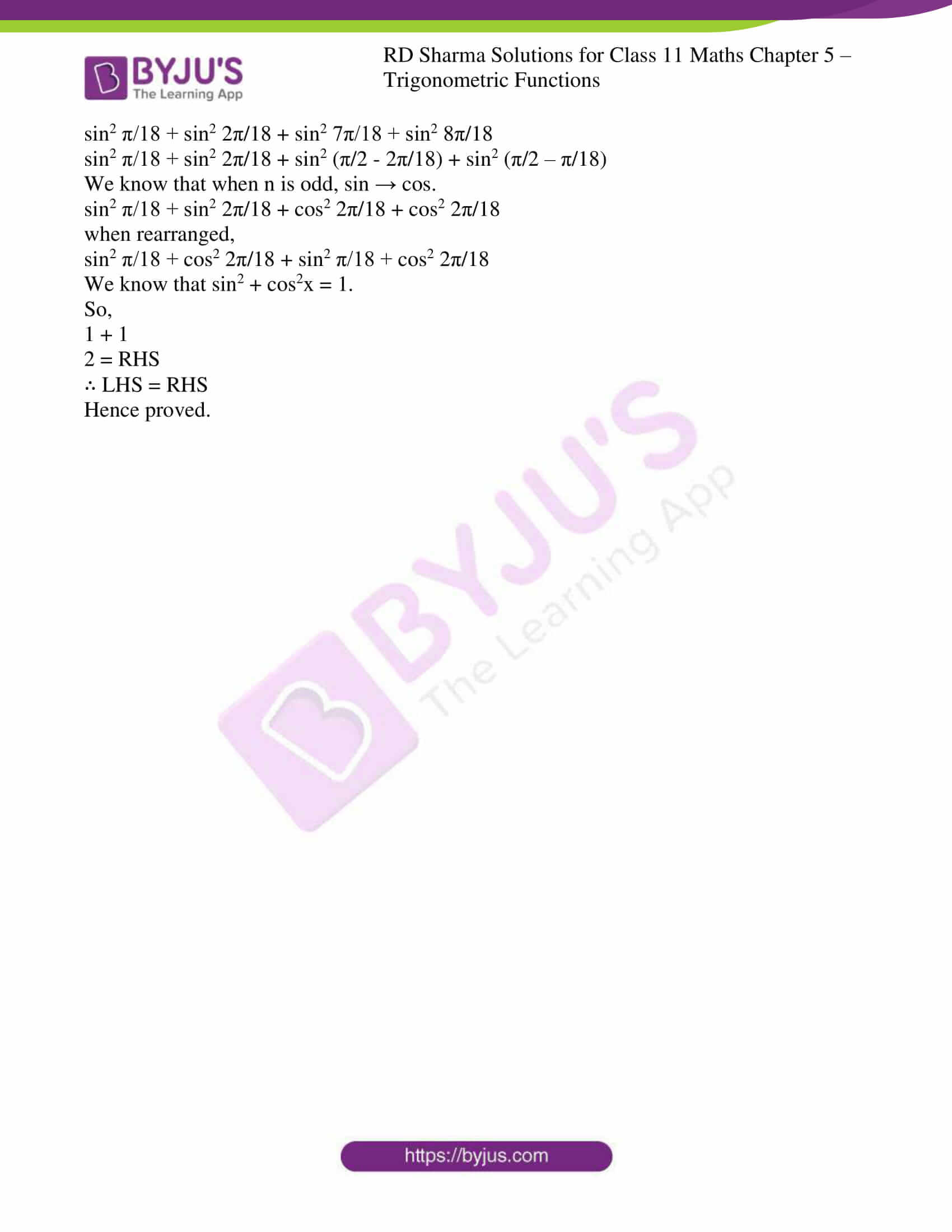Exercise 5.1

Exercise 5.2

Exercise 5.3

### Access answers to RD Sharma Solutions for Class 11 Maths Chapter 5 – Trigonometric Functions

EXERCISE 5.1 PAGE NO: 5.18

Prove the following identities:

1. sec4 x – sec2 x = tan4 x + tan2 x

Solution:

Let us consider LHS: sec4 x – sec2 x

(sec2 x)2 – sec2 x

By using the formula, sec2 θ = 1 + tan2 θ.

(1 + tan2 x) 2 – (1 + tan2 x)

1 + 2tan2 x + tan4 x – 1 – tan2 x

tan4 x + tan2 x

= RHS

∴ LHS = RHS

Hence proved.

2. sin6 x + cos6 x = 1 – 3 sin2 x cos2 x

Solution:

Let us consider LHS: sin6 x + cos6 x

(sin2 x) 3 + (cos2 x) 3

By using the formula, a3 + b3 = (a + b) (a2 + b2 – ab)

(sin2 x + cos2 x) [(sin2 x) 2 + (cos2 x) 2 – sin2 x cos2 x]

By using the formula, sin2 x + cos2 x = 1 and a2 + b2 = (a + b) 2 – 2ab

1 × [(sin2 x + cos2 x) 2 – 2sin2 x cos2 x – sin2 x cos2 x

12 – 3sin2 x cos2 x

1 – 3sin2 x cos2 x

= RHS

∴ LHS = RHS

Hence proved.

3. (cosec x – sin x) (sec x – cos x) (tan x + cot x) = 1

Solution:

Let us consider LHS: (cosec x – sin x) (sec x – cos x) (tan x + cot x)

By using the formulas

cosec θ = 1/sin θ;

sec θ = 1/cos θ;

tan θ = sin θ / cos θ;

cot θ = cos θ / sin θ

Now,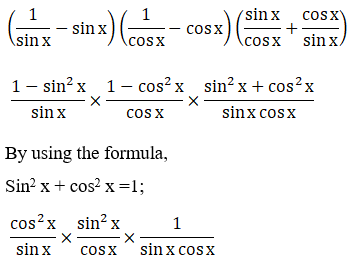1 = RHS

∴ LHS = RHS

Hence proved.

4. cosec x (sec x – 1) – cot x (1 – cos x) = tan x – sin x

Solution:

Let us consider LHS: cosec x (sec x – 1) – cot x (1 – cos x)

By using the formulas

cosec θ = 1/sin θ;

sec θ = 1/cos θ;

tan θ = sin θ / cos θ;

cot θ = cos θ / sin θ

Now,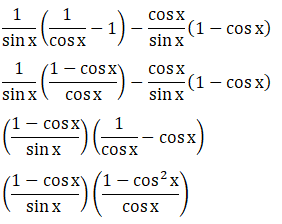By using the formula, 1 – cos2x = sin2x;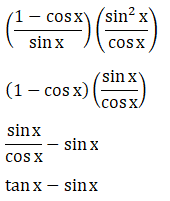= RHS

∴ LHS = RHS

Hence Proved.

5.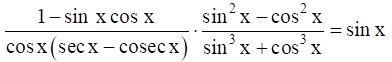Solution:

Let us consider the LHS: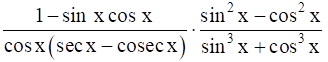By using the formula,

cosec θ = 1/sin θ;

sec θ = 1/cos θ;

Now,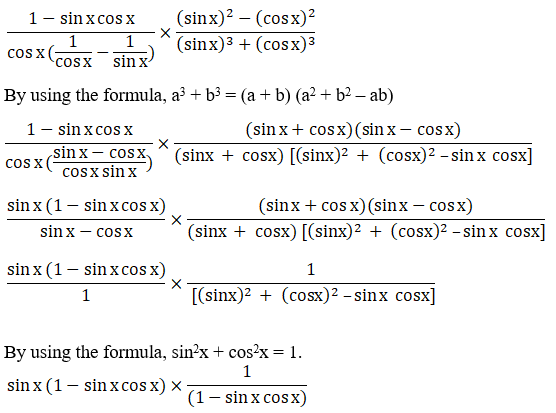sin x

= RHS

∴ LHS = RHS

Hence Proved.

6.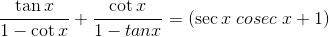Solution:

Let us consider the LHS: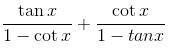By using the formula,

tan θ = sin θ / cos θ;

cot θ = cos θ / sin θ

Now,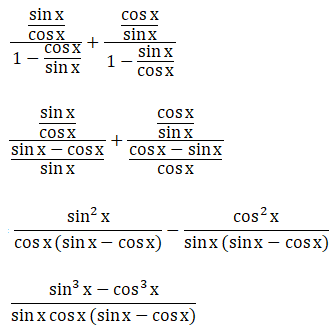By using the formula, a3 – b3 = (a – b) (a2 + b2 + ab)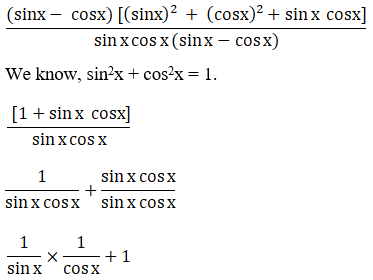By using the formula,

cosec θ = 1/sin θ,

sec θ = 1/cos θ;

cosec x × sec x + 1

sec x cosec x + 1

=RHS

∴ LHS = RHS

Hence Proved.

7.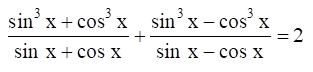Solution:

Let us consider LHS: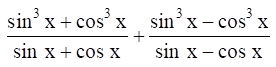By using the formula a3 ± b3 = (a ± b) (a2 + b2∓ ab)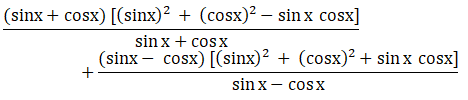We know, sin2x + cos2x = 1.

1 – sinx cosx + 1 + sinx cosx

2

= RHS

∴ LHS = RHS

Hence Proved.

8. (sec x sec y + tan x tan y)2 – (sec x tan y + tan x sec y)2 = 1

Solution:

Let us consider LHS:

(sec x sec y + tan x tan y)2 – (sec x tan y + tan x sec y)2

Expanding the above equation we get,

[(sec x sec y)2 + (tan x tan y)2 + 2 (sec x sec y) (tan x tan y)] – [(sec x tan y)2 + (tan x sec y)2 + 2 (sec x tan y) (tan x sec y)] [sec2 x sec2 y + tan2 x tan2 y + 2 (sec x sec y) (tan x tan y)] – [sec2 x tan2 y + tan2 x sec2 y + 2 (sec2 x tan2 y) (tan x sec y)]

sec2 x sec2 y – sec2 x tan2 y + tan2 x tan2 y – tan2 x sec2 y

sec2 x (sec2 y – tan2 y) + tan2 x (tan2 y – sec2 y)

sec2 x (sec2 y – tan2 y) – tan2 x (sec2 y – tan2 y)

We know, sec2 x – tan2 x = 1.

sec2 x × 1 – tan2 x × 1

sec2 x – tan2 x

1 = RHS

∴ LHS = RHS

Hence proved.

9.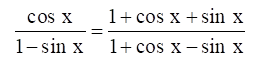Solution:

Let us Consider RHS: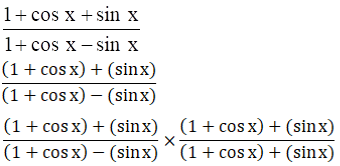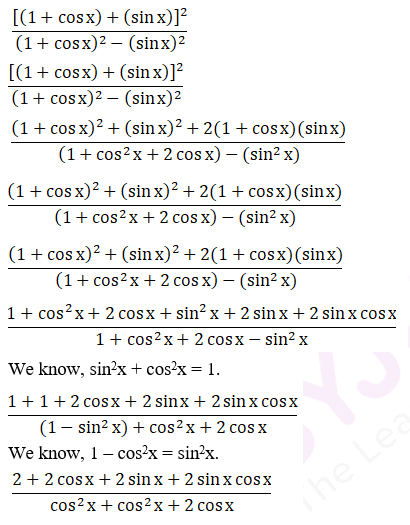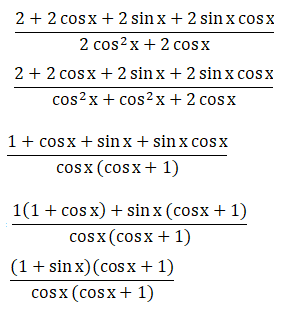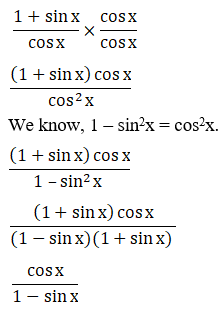= LHS

∴ LHS = RHS

Hence Proved.

10.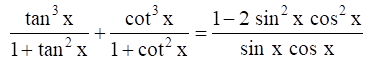Solution:

Let us consider LHS: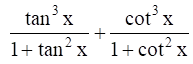By using the formulas,

1 + tan2x = sec2x and 1 + cot2x = cosec2x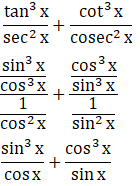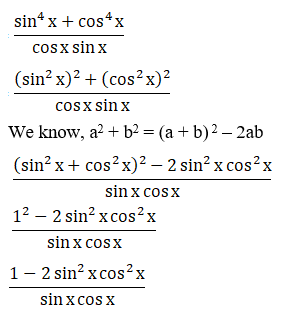= RHS

∴ LHS = RHS

Hence Proved.

11.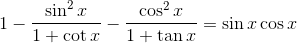Solution:

Let us consider LHS: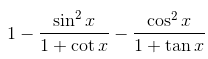By using the formula,

tan θ = sin θ / cos θ;

cot θ = cos θ / sin θ

Now,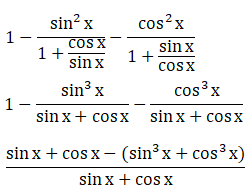By using the formula, a3 + b3 = (a + b) (a2 + b2– ab)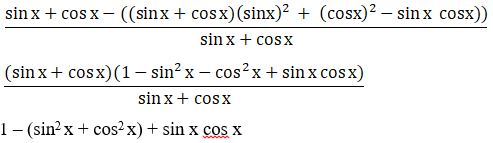We know, sin2 x + cos2 x = 1.

1 – 1 + sin x cos x

Sin x cos x

= RHS

∴ LHS = RHS

Hence proved.

12.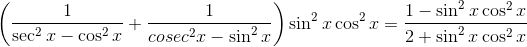Solution:

Let us consider LHS: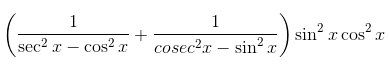By using the formula,

cosec θ = 1/sin θ,

sec θ = 1/cos θ;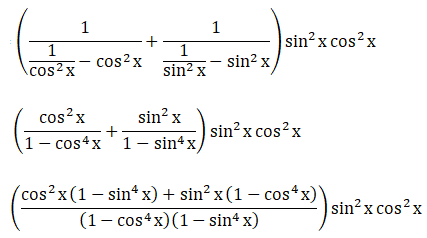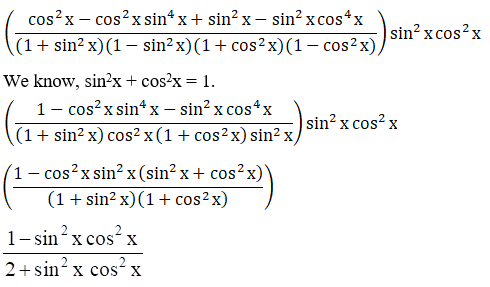= RHS

∴ LHS = RHS

Hence proved.

13. (1 + tan α tan β) 2 + (tan α – tan β) 2 = sec2 α sec2 β

Solution:

Let us consider LHS: (1 + tan α tan β) 2 + (tan α – tan β) 2

1+ tan2 α tan2 β + 2 tan α tan β + tan2 α + tan2 β – 2 tan α tan β

1 + tan2 α tan2 β + tan2 α + tan2 β

tan2 α (tan2 β + 1) + 1 (1 + tan2 β)

(1 + tan2 β) (1 + tan2 α)

We know, 1 + tan2 θ = sec2 θ

So,

sec2 α sec2 β

= RHS

∴ LHS = RHS

Hence proved.

EXERCISE 5.2 PAGE NO: 5.25

1. Find the values of the other five trigonometric functions in each of the following:

(i) cot x = 12/5, x in quadrant III

(ii) cos x = -1/2, x in quadrant II

(iii) tan x = 3/4, x in quadrant III

(iv) sin x = 3/5, x in quadrant I

Solution:

(i) cot x = 12/5, x in quadrant III

In third quadrant, tan x and cot x are positive. sin x, cos x, sec x, cosec x are negative.

By using the formulas,

tan x = 1/cot x

= 1/(12/5)

= 5/12

cosec x = –(1 + cot2 x)

= –(1 + (12/5)2)

= –(25+144)/25

= –(169/25)

= -13/5

sin x = 1/cosec x

= 1/(-13/5)

= -5/13

cos x = – (1 – sin2 x)

= – (1 – (-5/13)2)

= – √(169-25)/169

= – √(144/169)

= -12/13

sec x = 1/cos x

= 1/(-12/13)

= -13/12

∴ sin x = -5/13, cos x = -12/13, tan x = 5/12, cosec x = -13/5, sec x = -13/12

(ii) cos x = -1/2, x in quadrant II

In second quadrant, sin x and cosec x are positive. tan x, cot x, cos x, sec x are negative.

By using the formulas,

sin x = √(1 – cos2 x)

= √(1 – (-1/2)2)

= √(4-1)/4

= √(3/4)

= √3/2

tan x = sin x/cos x

= (√3/2)/(-1/2)

= -√3

cot x = 1/tan x

= 1/-√3

= -1/√3

cosec x = 1/sin x

= 1/(√3/2)

= 2/√3

sec x = 1/cos x

= 1/(-1/2)

= -2

∴ sin x = √3/2, tan x = -√3, cosec x = 2/√3, cot x = -1/√3 sec x = -2

(iii) tan x = 3/4, x in quadrant III

In third quadrant, tan x and cot x are positive. sin x, cos x, sec x, cosec x are negative.

By using the formulas,

sin x = √(1 – cos2 x)

= – √(1-(-4/5)2)

= – √(25-16)/25

= – √(9/25)

= – 3/5

cos x = 1/sec x

= 1/(-5/4)

= -4/5

cot x = 1/tan x

= 1/(3/4)

= 4/3

cosec x = 1/sin x

= 1/(-3/5)

= -5/3

sec x = -√(1 + tan2 x)

= – √(1+(3/4)2)

= – √(16+9)/16

= – √ (25/16)

= -5/4

∴ sin x = -3/5, cos x = -4/5, cosec x = -5/3, sec x = -5/4, cot x = 4/3

(iv) sin x = 3/5, x in quadrant I

In first quadrant, all trigonometric ratios are positive.

So, by using the formulas,

tan x = sin x/cos x

= (3/5)/(4/5)

= 3/4

cosec x = 1/sin x

= 1/(3/5)

= 5/3

cos x = √(1-sin2 x)

= √(1 – (-3/5)2)

= √(25-9)/25

= √(16/25)

= 4/5

sec x = 1/cos x

= 1/(4/5)

= 5/4

cot x = 1/tan x

= 1/(3/4)

= 4/3

∴ cos x = 4/5, tan x = 3/4, cosec x = 5/3, sec x = 5/4, cot x = 4/3

2. If sin x = 12/13 and lies in the second quadrant, find the value of sec x + tan x.

Solution:

Given:

Sin x = 12/13 and x lies in the second quadrant.

We know, in second quadrant, sin x and cosec x are positive and all other ratios are negative.

By using the formulas,

Cos x = √(1-sin2 x)

= – √(1-(12/13)2)

= – √(1- (144/169))

= – √(169-144)/169

= -√(25/169)

= – 5/13

We know,

tan x = sin x/cos x

sec x = 1/cos x

Now,

tan x = (12/13)/(-5/13)

= -12/5

sec x = 1/(-5/13)

= -13/5

Sec x + tan x = -13/5 + (-12/5)

= (-13-12)/5

= -25/5

= -5

∴ Sec x + tan x = -5

3. If sin x = 3/5, tan y = 1/2 and π/2 < x< π< y< 3π/2 find the value of 8 tan x -√5 sec y.

Solution:

Given:

sin x = 3/5, tan y = 1/2 and π/2 < x< π< y< 3π/2

We know that, x is in second quadrant and y is in third quadrant.

In second quadrant, cos x and tan x are negative.

In third quadrant, sec y is negative.

By using the formula,

cos x = – √(1-sin2 x)

tan x = sin x/cos x

Now,

cos x = – √(1-sin2 x)

= – √(1 – (3/5)2)

= – √(1 – 9/25)

= – √((25-9)/25)

= – √(16/25)

= – 4/5

tan x = sin x/cos x

= (3/5)/(-4/5)

= 3/5 × -5/4

= -3/4

We know that sec y = – √(1+tan2 y)

= – √(1 + (1/2)2)

= – √(1 + 1/4)

= – √((4+1)/4)

= – √(5/4)

= – √5/2

Now, 8 tan x – √5 sec y = 8(-3/4) – √5(-√5/2)

= -6 + 5/2

= (-12+5)/2

= -7/2

∴ 8 tan x – √5 sec y = -7/2

4. If sin x + cos x = 0 and x lies in the fourth quadrant, find sin x and cos x.

Solution:

Given:

Sin x + cos x = 0 and x lies in fourth quadrant.

Sin x = -cos x

Sin x/cos x = -1

So, tan x = -1 (since, tan x = sin x/cos x)

We know that, in fourth quadrant, cos x and sec x are positive and all other ratios are negative.

By using the formulas,

Sec x = √(1 + tan2 x)

Cos x = 1/sec x

Sin x = – √(1- cos2 x)

Now,

Sec x = √(1 + tan2 x)

= √(1 + (-1)2)

= √2

Cos x = 1/sec x

= 1/√2

Sin x = – √(1 – cos2 x)

= – √(1 – (1/√2)2)

= – √(1 – (1/2))

= – √((2-1)/2)

= – √(1/2)

= -1/√2

∴ sin x = -1/√2 and cos x = 1/√2

5. If cos x = -3/5 and π<x<3π/2 find the values of other five trigonometric functions and hence evaluate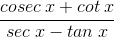Solution:

Given:

cos x= -3/5 and π <x < 3π/2

We know that in the third quadrant, tan x and cot x are positive and all other rations are negative.

By using the formulas,

Sin x = – √(1-cos2 x)

Tan x = sin x/cos x

Cot x = 1/tan x

Sec x = 1/cos x

Cosec x = 1/sin x

Now,

Sin x = – √(1-cos2 x)

= – √(1-(-3/5)2)

= – √(1-9/25)

= – √((25-9)/25)

= – √(16/25)

= – 4/5

Tan x = sin x/cos x

= (-4/5)/(-3/5)

= -4/5 × -5/3

= 4/3

Cot x = 1/tan x

= 1/(4/3)

= 3/4

Sec x = 1/cos x

= 1/(-3/5)

= -5/3

Cosec x = 1/sin x

= 1/(-4/5)

= -5/4= [(-5/4) + (3/4)] / [(-5/3) – (4/3)]

= [(-5+3)/4] / [(-5-4)/3]

= [-2/4] / [-9/3]

= [-1/2] / [-3]

= 1/6

EXERCISE 5.3 PAGE NO: 5.39

1. Find the values of the following trigonometric ratios:

(i) sin 5π/3

(ii) sin 17π

(iii) tan 11π/6

(iv) cos (-25π/4)

(v) tan 7π/4

(vi) sin 17π/6

(vii) cos 19π/6

(viii) sin (-11π/6)

(ix) cosec (-20π/3)

(x) tan (-13π/4)

(xi) cos 19π/4

(xii) sin 41π/4

(xiii) cos 39π/4

(xiv) sin 151π/6

Solution:

(i) sin 5π/3

5π/3 = (5π/3 × 180)o

= 300o

= (90×3 + 30)o

Since, 300o lies in IV quadrant in which sine function is negative.

sin 5π/3 = sin (300)o

= sin (90×3 + 30)o

= – cos 30o

= – √3/2

(ii) sin 17π

Sin 17π = sin 3060o

= sin (90×34 + 0)o

Since, 3060o lies in the negative direction of x-axis i.e., on boundary line of II and III quadrants.

Sin 17π = sin (90×34 + 0)o

= – sin 0o

= 0

(iii) tan 11π/6

tan 11π/6 = (11/6 × 180)o

= 330o

Since, 330o lies in the IV quadrant in which tangent function is negative.

tan 11π/6 = tan (300)o

= tan (90×3 + 60)o

= – cot 60o

= – 1/√3

(iv) cos (-25π/4)

cos (-25π/4) = cos (-1125)o

= cos (1125)o

Since, 1125o lies in the I quadrant in which cosine function is positive.

cos (1125)o = cos (90×12 + 45)o

= cos 45o

= 1/√2

(v) tan 7π/4

tan 7π/4 = tan 315o

= tan (90×3 + 45)o

Since, 315o lies in the IV quadrant in which tangent function is negative.

tan 315o = tan (90×3 + 45)o

= – cot 45o

= -1

(vi) sin 17π/6

sin 17π/6 = sin 510o

= sin (90×5 + 60)o

Since, 510o lies in the II quadrant in which sine function is positive.

sin 510o = sin (90×5 + 60)o

= cos 60o

= 1/2

(vii) cos 19π/6

cos 19π/6 = cos 570o

= cos (90×6 + 30)o

Since, 570o lies in III quadrant in which cosine function is negative.

cos 570o = cos (90×6 + 30)o

= – cos 30o

= – √3/2

(viii) sin (-11π/6)

sin (-11π/6) = sin (-330o)

= – sin (90×3 + 60)o

Since, 330o lies in the IV quadrant in which the sine function is negative.

sin (-330o) = – sin (90×3 + 60)o

= – (-cos 60o)

= – (-1/2)

= 1/2

(ix) cosec (-20π/3)

cosec (-20π/3) = cosec (-1200)o

= – cosec (1200)o

= – cosec (90×13 + 30)o

Since, 1200o lies in the II quadrant in which cosec function is positive.

cosec (-1200)o = – cosec (90×13 + 30)o

= – sec 30o

= -2/√3

(x) tan (-13π/4)

tan (-13π/4) = tan (-585)o

= – tan (90×6 + 45)o

Since, 585o lies in the III quadrant in which the tangent function is positive.

tan (-585)o = – tan (90×6 + 45)o

= – tan 45o

= -1

(xi) cos 19π/4

cos 19π/4 = cos 855o

= cos (90×9 + 45)o

Since, 855o lies in the II quadrant in which the cosine function is negative.

cos 855o = cos (90×9 + 45)o

= – sin 45o

= – 1/√2

(xii) sin 41π/4

sin 41π/4 = sin 1845o

= sin (90×20 + 45)o

Since, 1845o lies in the I quadrant in which the sine function is positive.

sin 1845o = sin (90×20 + 45)o

= sin 45o

= 1/√2

(xiii) cos 39π/4

cos 39π/4 = cos 1755o

= cos (90×19 + 45)o

Since, 1755o lies in the IV quadrant in which the cosine function is positive.

cos 1755o = cos (90×19 + 45)o

= sin 45o

= 1/√2

(xiv) sin 151π/6

sin 151π/6 = sin 4530o

= sin (90×50 + 30)o

Since, 4530o lies in the III quadrant in which the sine function is negative.

sin 4530o = sin (90×50 + 30)o

= – sin 30o

= -1/2

2. prove that:
(i) tan 225o cot 405o + tan 765o cot 675o = 0

(ii) sin 8π/3 cos 23π/6 + cos 13π/3 sin 35π/6 = 1/2

(iii) cos 24o + cos 55o + cos 125o + cos 204o + cos 300o = 1/2

(iv) tan (-125o) cot (-405o) – tan (-765o) cot (675o) = 0

(v) cos 570o sin 510o + sin (-330o) cos (-390o) = 0

(vi) tan 11π/3 – 2 sin 4π/6 – 3/4 cosec2 π/4 + 4 cos2 17π/6 = (3 – 4√3)/2

(vii) 3 sin π/6 sec π/3 – 4 sin 5π/6 cot π/4 = 1

Solution:

(i) tan 225o cot 405o + tan 765o cot 675o = 0

Let us consider LHS:

tan 225° cot 405° + tan 765° cot 675°

tan (90° × 2 + 45°) cot (90° × 4 + 45°) + tan (90° × 8 + 45°) cot (90° × 7 + 45°)

We know that when n is odd, cot → tan.

tan 45° cot 45° + tan 45° [-tan 45°]

tan 45° cot 45° – tan 45° tan 45°

1 × 1 – 1 × 1

1 – 1

0 = RHS

∴ LHS = RHS

Hence proved.

(ii) sin 8π/3 cos 23π/6 + cos 13π/3 sin 35π/6 = 1/2

Let us consider LHS:

sin 8π/3 cos 23π/6 + cos 13π/3 sin 35π/6

sin 480° cos 690° + cos 780° sin 1050°

sin (90° × 5 + 30°) cos (90° × 7 + 60°) + cos (90° × 8 + 60°) sin (90° × 11 + 60°)

We know that when n is odd, sin → cos and cos → sin.

cos 30° sin 60° + cos 60° [-cos 60°]

√3/2 × √3/2 – 1/2 × 1/2

3/4 – 1/4

2/4

1/2

= RHS

∴ LHS = RHS

Hence proved.

(iii) cos 24o + cos 55o + cos 125o + cos 204o + cos 300o = 1/2

Let us consider LHS:

cos 24o + cos 55o + cos 125o + cos 204o + cos 300o

cos 24° + cos (90° × 1 – 35°) + cos (90° × 1 + 35°) + cos (90° × 2 + 24°) + cos (90° × 3 + 30°)

We know that when n is odd, cos → sin.

cos 24° + sin 35° – sin 35° – cos 24° + sin 30°

0 + 0 + 1/2

1/2

= RHS

∴ LHS = RHS

Hence proved.

(iv) tan (-125o) cot (-405o) – tan (-765o) cot (675o) = 0

Let us consider LHS:

tan (-125o) cot (-405o) – tan (-765o) cot (675o)

We know that tan (-x) = -tan (x) and cot (-x) = -cot (x).

[-tan (225°)] [-cot (405°)] – [-tan (765°)] cot (675°)

tan (225°) cot (405°) + tan (765°) cot (675°)

tan (90° × 2 + 45°) cot (90° × 4 + 45°) + tan (90° × 8 + 45°) cot (90° × 7 + 45°)

tan 45° cot 45° + tan 45° [-tan 45°]

1 × 1 + 1 × (-1)

1 – 1

0

= RHS

∴ LHS = RHS

Hence proved.

(v) cos 570o sin 510o + sin (-330o) cos (-390o) = 0

Let us consider LHS:

cos 570o sin 510o + sin (-330o) cos (-390o)

We know that sin (-x) = -sin (x) and cos (-x) = +cos (x).

cos 570o sin 510o + [-sin (330o)] cos (390o)

cos 570o sin 510o – sin (330o) cos (390o)

cos (90° × 6 + 30°) sin (90° × 5 + 60°) – sin (90° × 3 + 60°) cos (90° × 4 + 30°)

We know that cos is negative at 90° + θ i.e. in Q2 and when n is odd, sin → cos and cos → sin.

-cos 30° cos 60° – [-cos 60°] cos 30°

-cos 30° cos 60° + cos 60° cos 30°

0

= RHS

∴ LHS = RHS

Hence proved.

(vi) tan 11π/3 – 2 sin 4π/6 – 3/4 cosec2 π/4 + 4 cos2 17π/6 = (3 – 4√3)/2

Let us consider LHS:

tan 11π/3 – 2 sin 4π/6 – 3/4 cosec2 π/4 + 4 cos2 17π/6

tan (11 × 180o)/3 – 2 sin (4 × 180o)/6 – 3/4 cosec2 180o/4 + 4 cos2 (17 × 180o)/6

tan 660o – 2 sin 120o – 3/4 (cosec 45o)2 + 4 (cos 510o)2

tan (90° × 7 + 30°) – 2 sin (90° × 1 + 30°) – 3/4 [cosec 45°]2 + 4 [cos (90° × 5 + 60°)]2

We know that tan and cos is negative at 90° + θ i.e. in Q2 and when n is odd, tan → cot, sin → cos and cos → sin.

[-cot 30°] – 2 cos 30° – 3/4 [cosec 45°]2 + [-sin 60°]2

– cot 30° – 2 cos 30° – 3/4 [cosec 45°]2 + [sin 60°]2

-√3 – 2√3/2 – 3/4 (√2)2 + 4 (√3/2)2

-√3 – √3 – 6/4 + 12/4

(3 – 4√3)/2

= RHS

∴ LHS = RHS

Hence proved.

(vii) 3 sin π/6 sec π/3 – 4 sin 5π/6 cot π/4 = 1

Let us consider LHS:

3 sin π/6 sec π/3 – 4 sin 5π/6 cot π/4

3 sin 180o/6 sec 180o/3 – 4 sin 5(180o)/6 cot 180o/4

3 sin 30° sec 60° – 4 sin 150° cot 45°

3 sin 30° sec 60° – 4 sin (90° × 1 + 60°) cot 45°

We know that when n is odd, sin → cos.

3 sin 30° sec 60° – 4 cos 60° cot 45°

3 (1/2) (2) – 4 (1/2) (1)

3 – 2

1

= RHS

∴ LHS = RHS

Hence proved.

3. Prove that:

(i)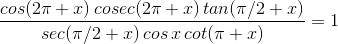(ii)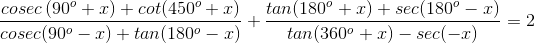(iii)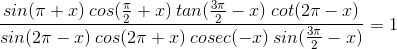(iv)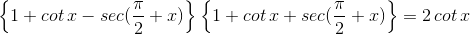(v)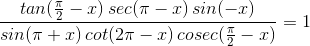Solution:

(i)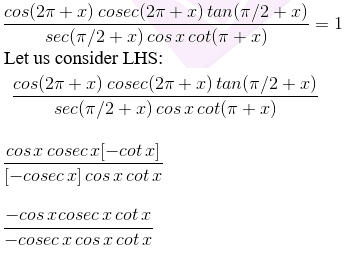1 = RHS

∴ LHS = RHS

Hence proved.

(ii)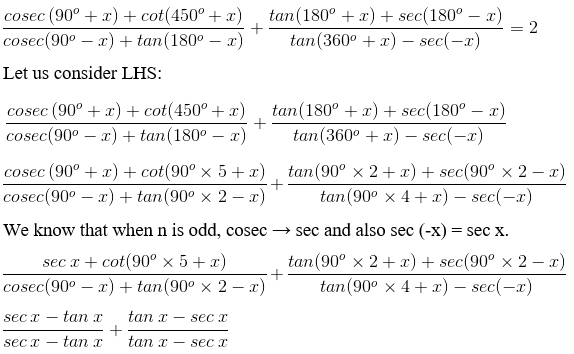1 + 1

2 = RHS

∴ LHS = RHS

Hence proved.

(iii)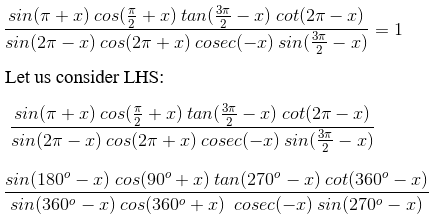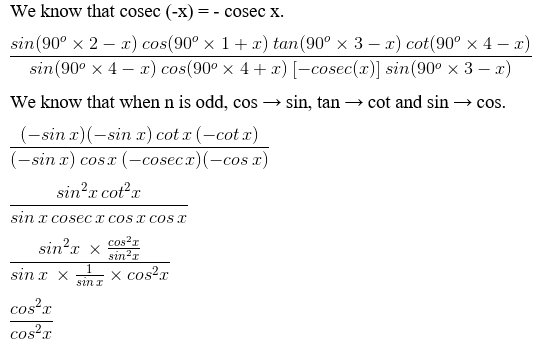1 = RHS

∴ LHS = RHS

Hence proved.

(iv)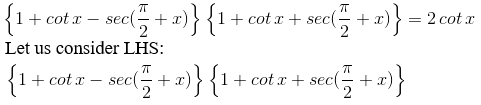{1 + cot x – (-cosec x)} {1 + cot x + (-cosec x)}

{1 + cot x + cosec x} {1 + cot x – cosec x}

{(1 + cot x) + (cosec x)} {(1 + cot x) – (cosec x)}

By using the formula, (a + b) (a – b) = a2 – b2

(1 + cot x)2 – (cosec x)2

1 + cot2 x + 2 cot x – cosec2 x

We know that 1 + cot2 x = cosec2 x

cosec2 x + 2 cot x – cosec2 x

2 cot x = RHS

∴ LHS = RHS

Hence proved.

(v)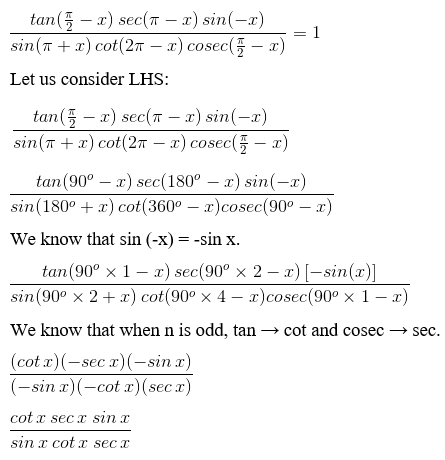1 = RHS

∴ LHS = RHS

Hence proved.

4. Prove that: sin2 π/18 + sin2 π/9 + sin2 7π/18 + sin2 4π/9 = 2

Solution:

Let us consider LHS:

sin2 π/18 + sin2 π/9 + sin2 7π/18 + sin2 4π/9

sin2 π/18 + sin2 2π/18 + sin2 7π/18 + sin2 8π/18

sin2 π/18 + sin2 2π/18 + sin2 (π/2 – 2π/18) + sin2 (π/2 – π/18)

We know that when n is odd, sin → cos.

sin2 π/18 + sin2 2π/18 + cos2 2π/18 + cos2 2π/18

when rearranged,

sin2 π/18 + cos2 2π/18 + sin2 π/18 + cos2 2π/18

We know that sin2 + cos2x = 1.

So,

1 + 1

2 = RHS

∴ LHS = RHS

Hence proved.

## Frequently Asked Questions on RD Sharma Solutions for Class 11 Maths Chapter 5

### How RD Sharma Solutions for Class 11 Maths Chapter 5 helpful for board exams?

RD Sharma Solutions for Class 11 Maths Chapter 5 provides answers with detailed descriptions as per term limit particularised by the Board for self-evaluation. Solving these solutions will provide excellent practice for the students so they can finish the paper on time. So, it’s clear that the RD Sharma Solutions for Class 11 Maths Chapter 5 are essential to score high in examinations. Students can get acquainted with writing exams and will be able to face exams more confidently.

### Mention the topics that are covered in RD Sharma Solutions for Class 11 Maths Chapter 5?

The topics that are covered in RD Sharma Solutions for Class 11 Maths Chapter 5 are
Trigonometric functions of a real number.
Values of trigonometric functions.
Trigonometric identities.
Fundamental trigonometric identities.
Signs of trigonometric functions.
Variations in values of trigonometric functions in different quadrants.
Values of trigonometric functions at allied angles.
Periodic functions.
Even and odd functions.
By learning these RD Sharma solutions thoroughly students will be able to solve complex problems easily.

### Is it necessary to practice all the exercises present in Chapter 5 of RD Sharma Solutions for Class 11 Maths?

Yes, it is compulsory to practice all the exercises present in Chapter 5 of RD Sharma Solutions for Class 11 Maths. Because all exercises contain numerous questions to solve which may come in their finals. This makes them more confident towards the syllabus they have. The expert faculty at BYJU’S provides students with PDF of solutions to solve the problems according to the CBSE syllabus. The solutions for exercise wise problems are prepared after vast research is conducted on each topic.